Teachoo Questions

Class 10
Chapter 12 Class 10 - Electricity

The password to open the file is -   teachooisbest

## A current of 1 A is drawn by a filament of an electric bulb. Number of electrons passing through a cross section of the filament in 16 seconds would be roughly

(a) 10 20

(b) 10 16

(c) 10 18

(d) 10 23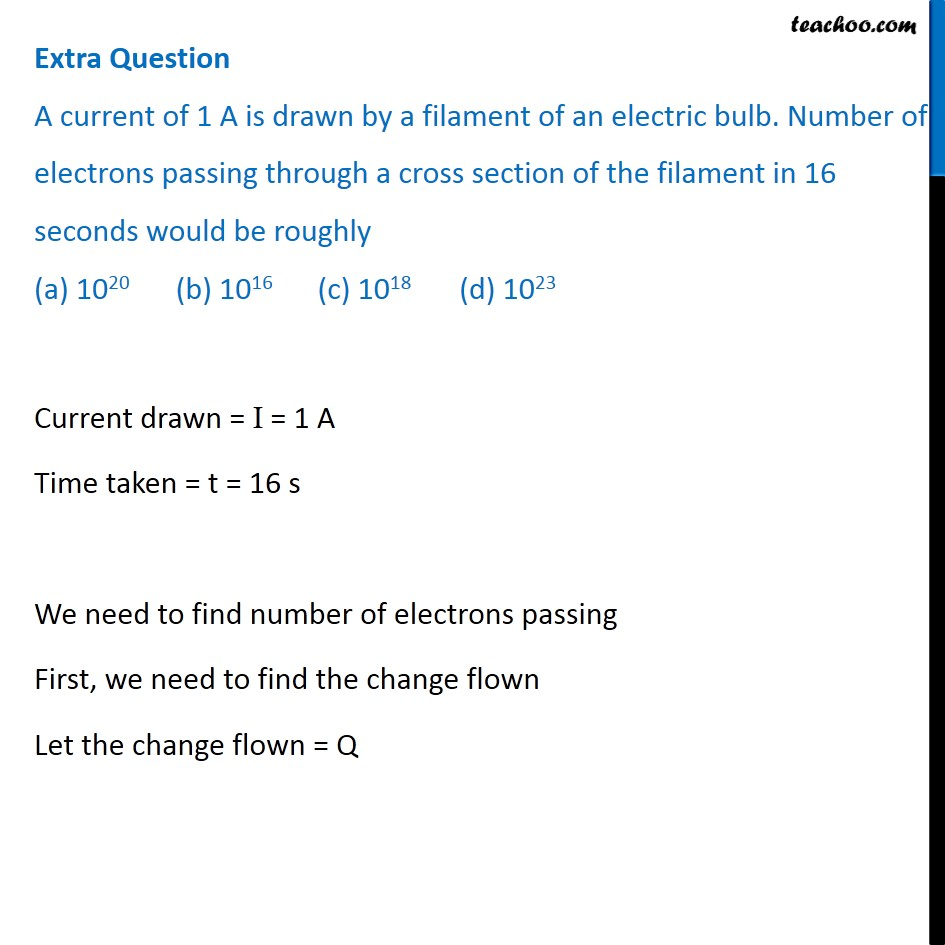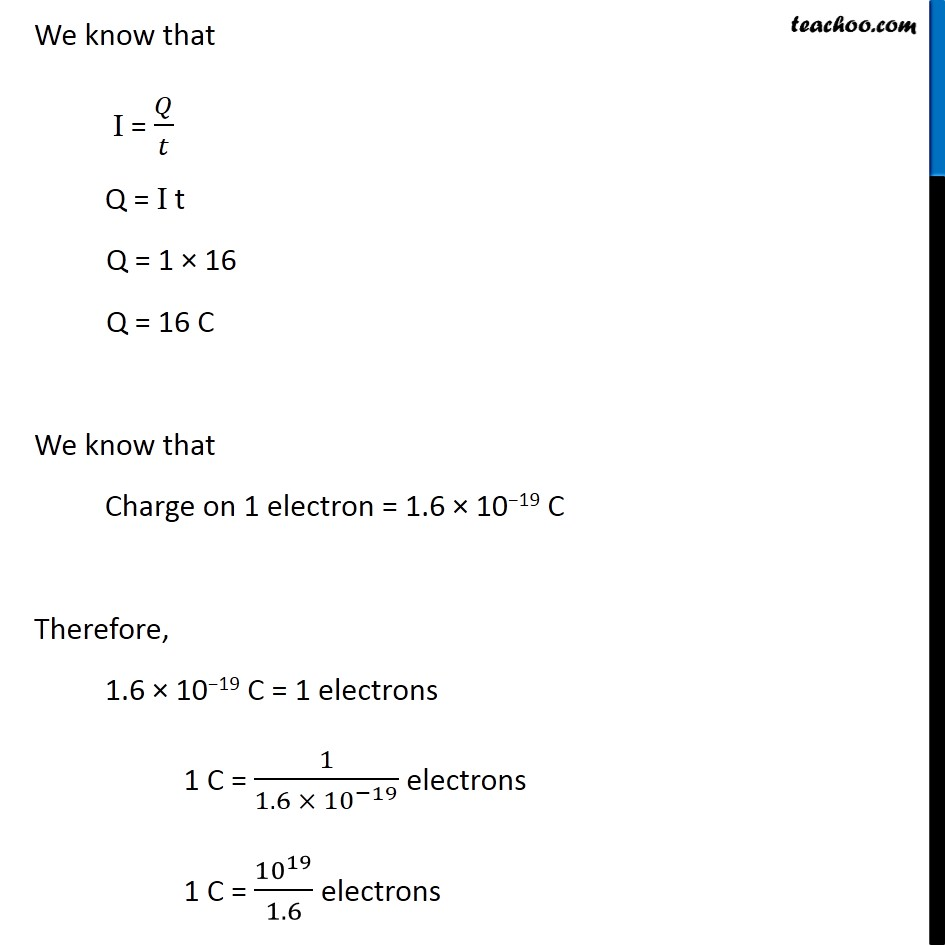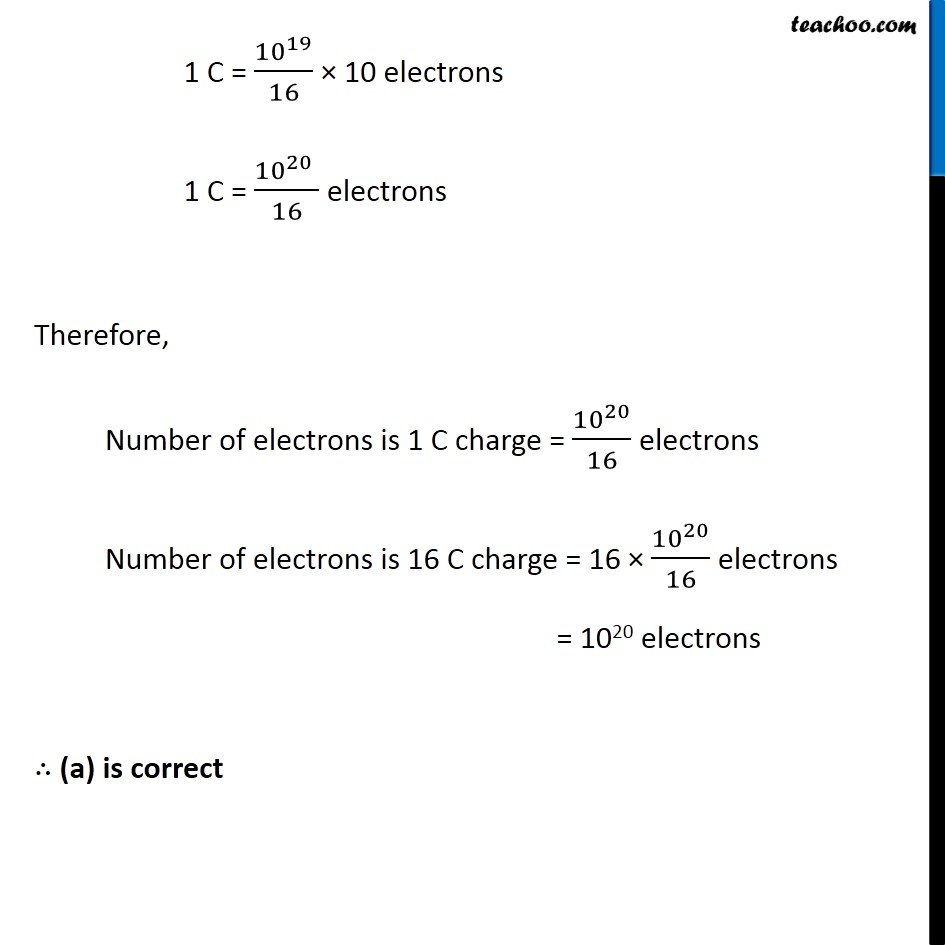## What is the maximum resistance which can be made using five resistors each of 1/5 Ω?

(a) 1/5 Ω

(b) 10 Ω

(c) 5 Ω

(d) 1 Ω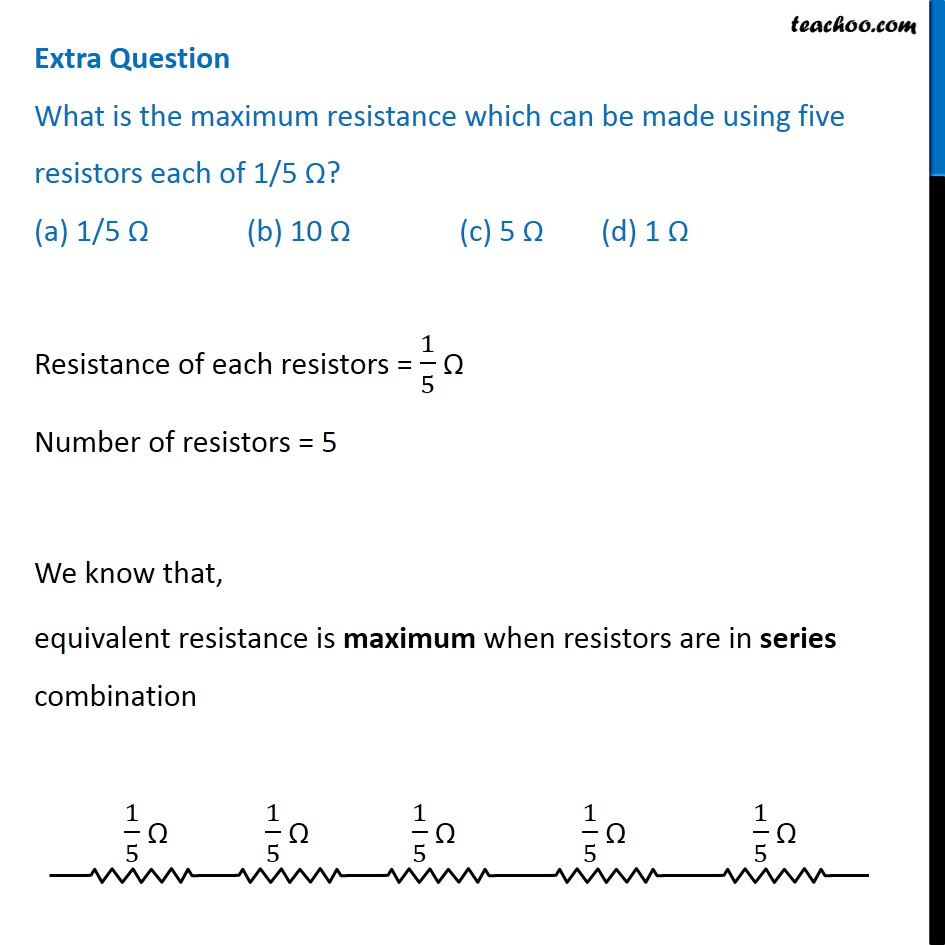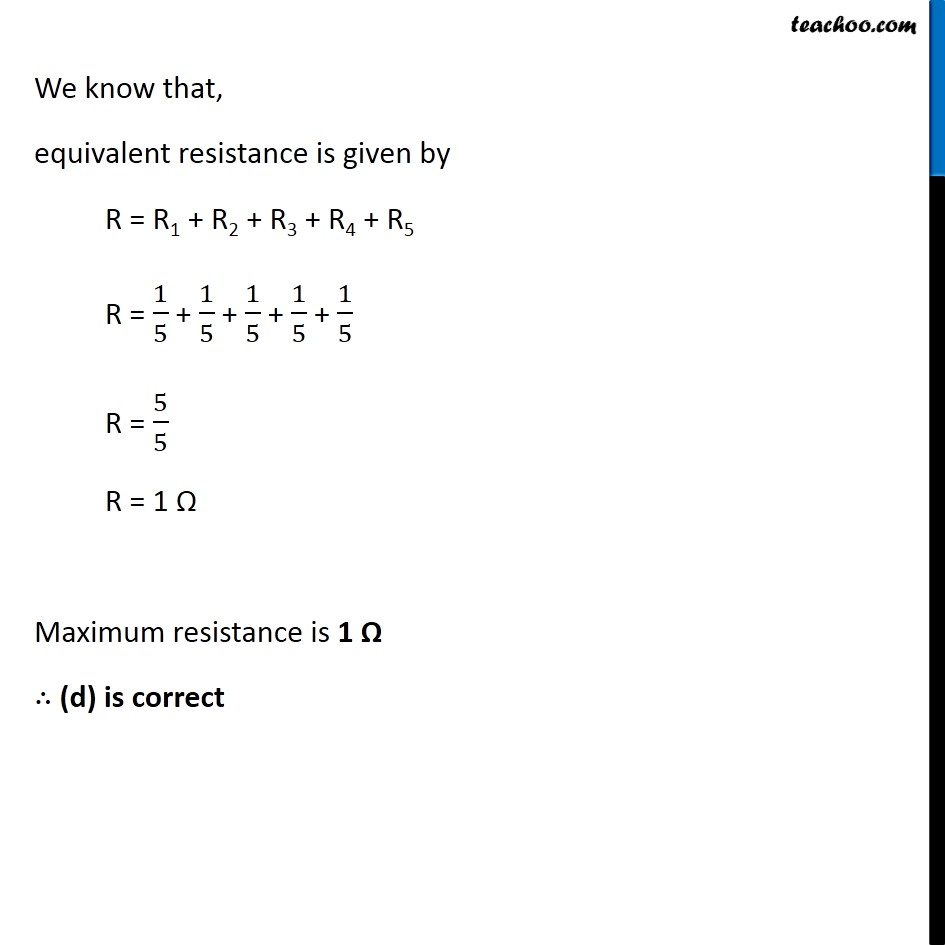## What is the minimum resistance which can be made using five resistors each of 1/5 Ω?

(a) 1/5 Ω

(b) 1/25 Ω

(c) 1/10 Ω

(d) 25 Ω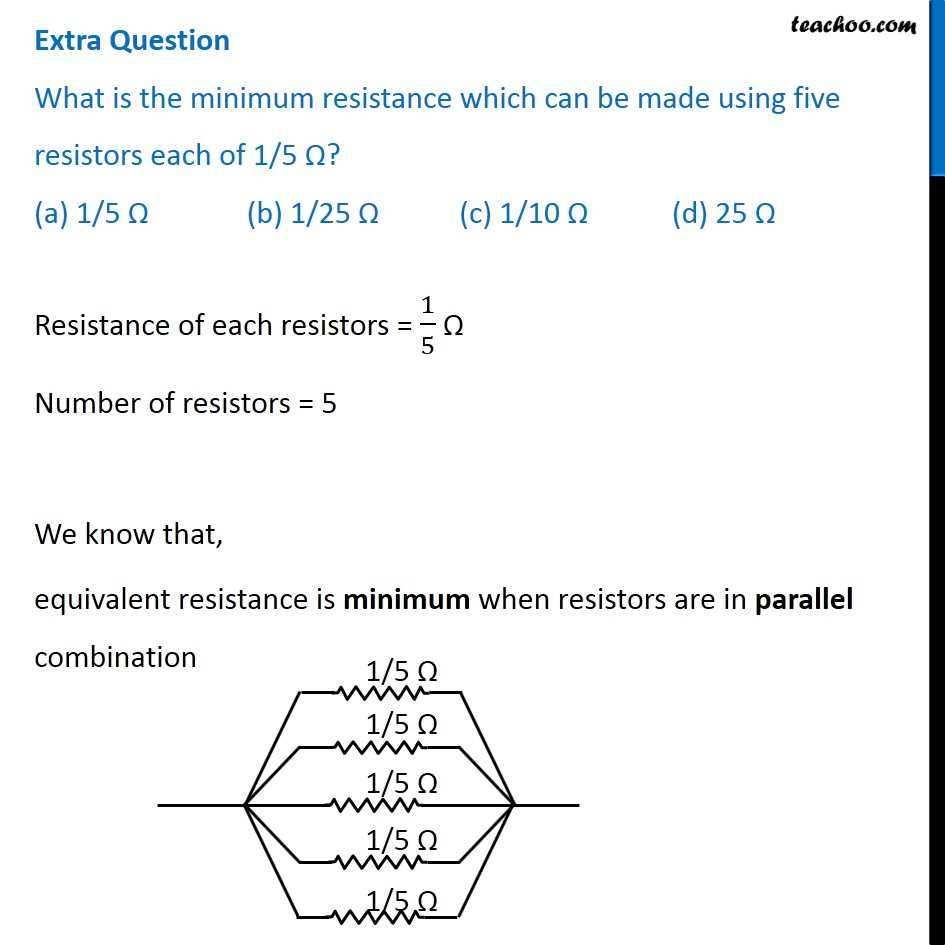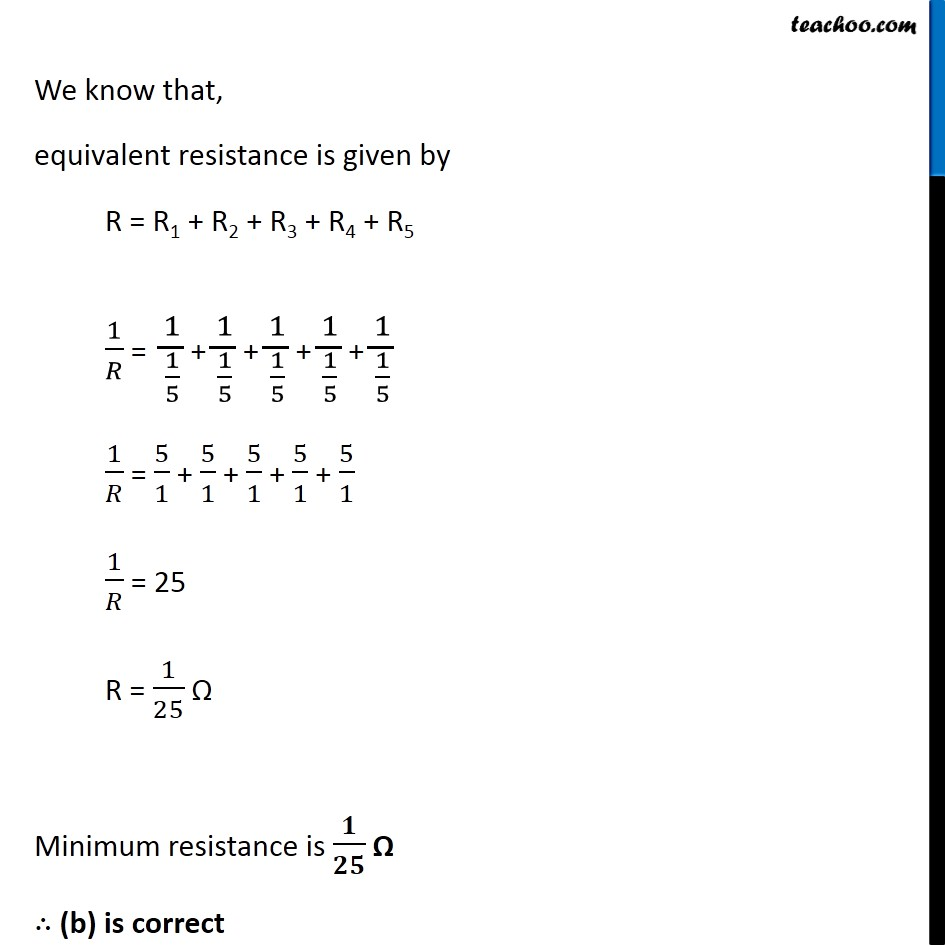## A cylindrical conductor of length l and uniform area of cross section A has resistance R. Another conductor of length 2l and resistance R of the same material has area of cross section

(a) A/2

(b) 3A/2

(c) 2A

(d) 3A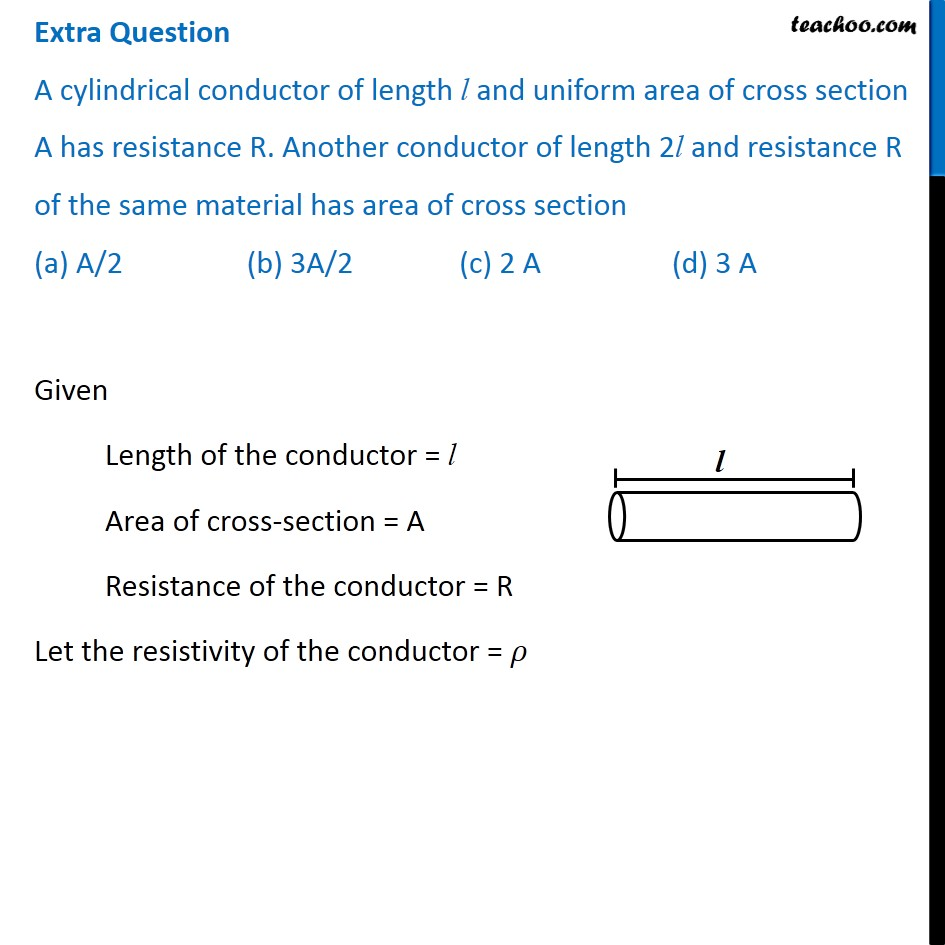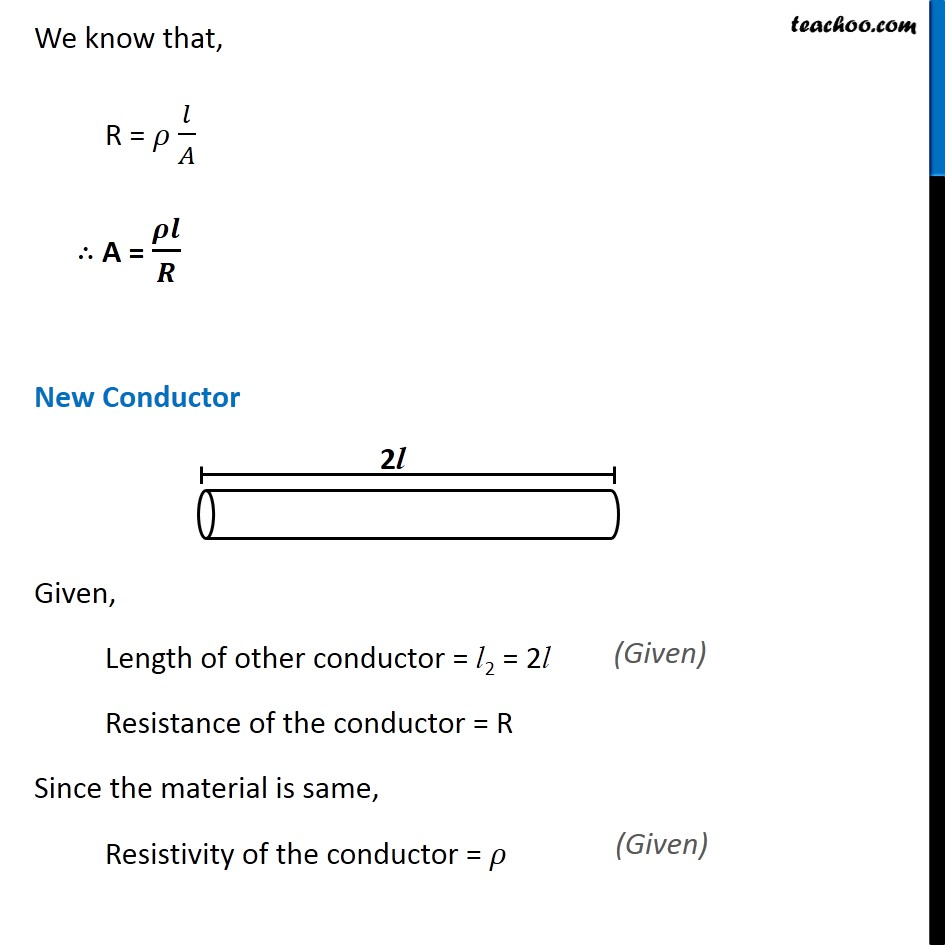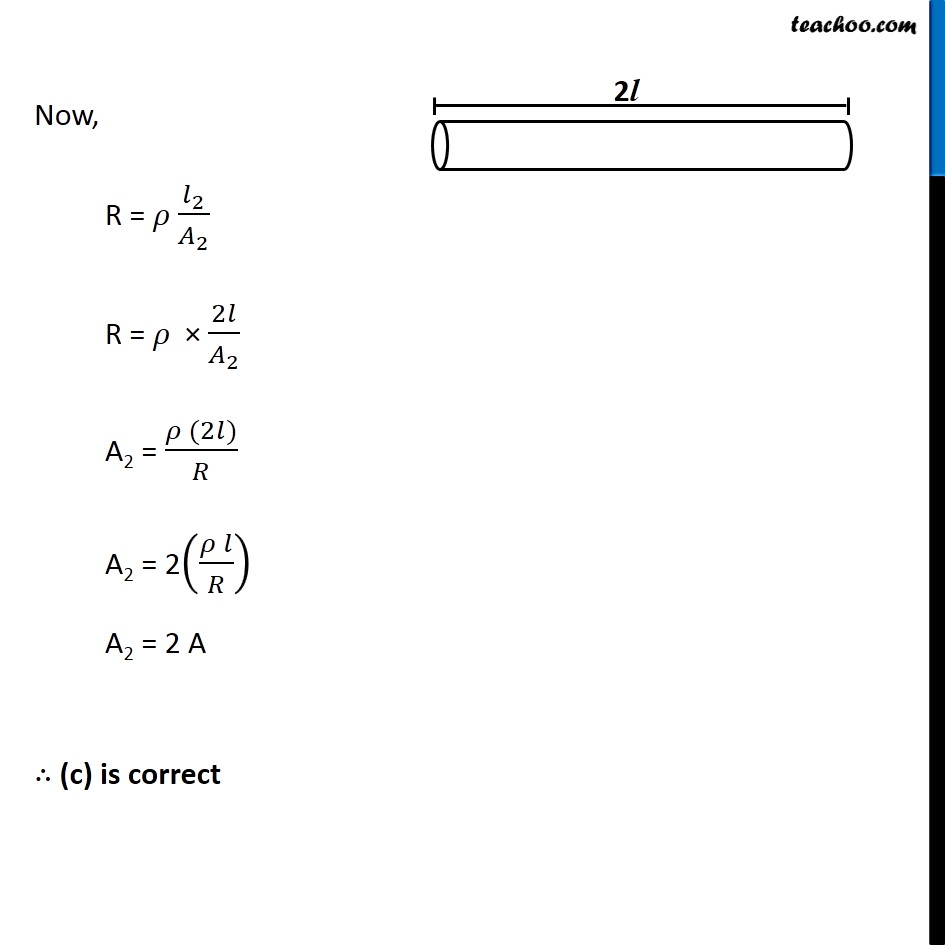## The resistivity does not change if

(a) the material is changed

(b) the temperature is changed

(c) the shape of the resistor is changed

(d) both material and temperature are changed

the shape of the resistor is changed

Explanation - The resistivity of a substance depends on the nature of the material and the physical conditions like temperature.
It does not depend on the shape of the resistor.

## In an electrical circuit two resistors of 2 Ω and 4 Ω respectively are connected in series to a 6 V battery. The heat dissipated by the 4 Ω resistor in 5 s will be

(a) 5 J

(b) 10 J

(c) 20 J

(d) 30 J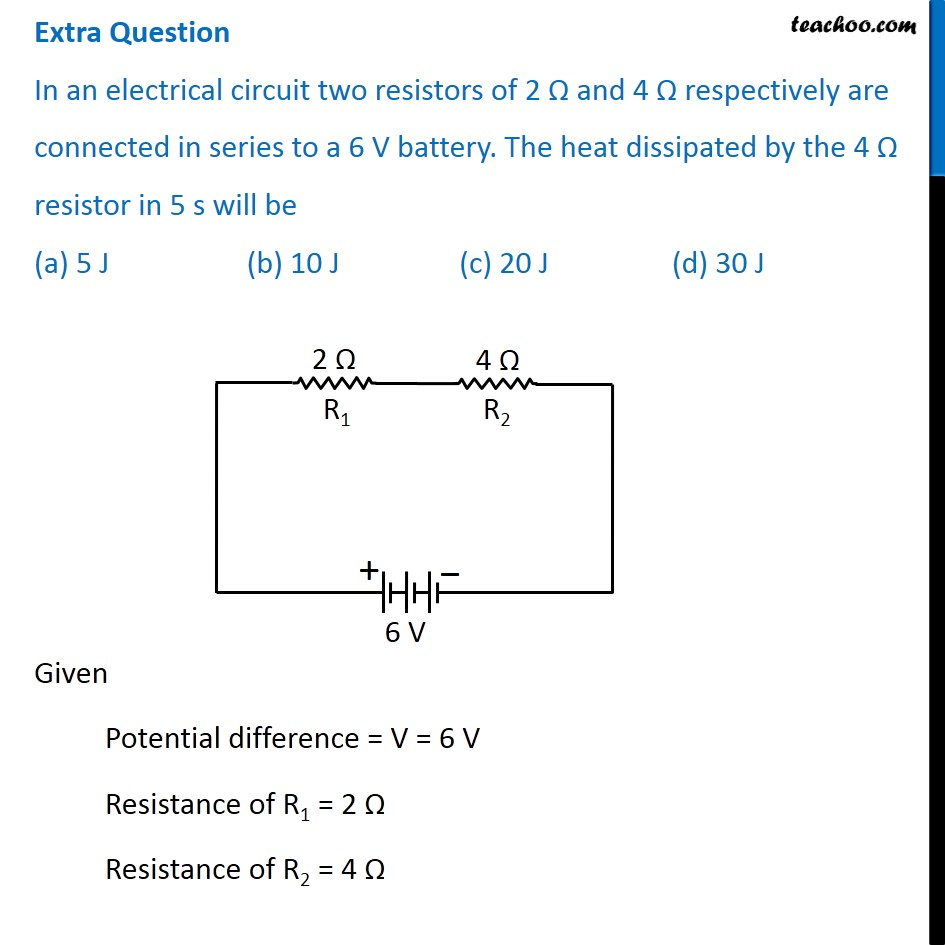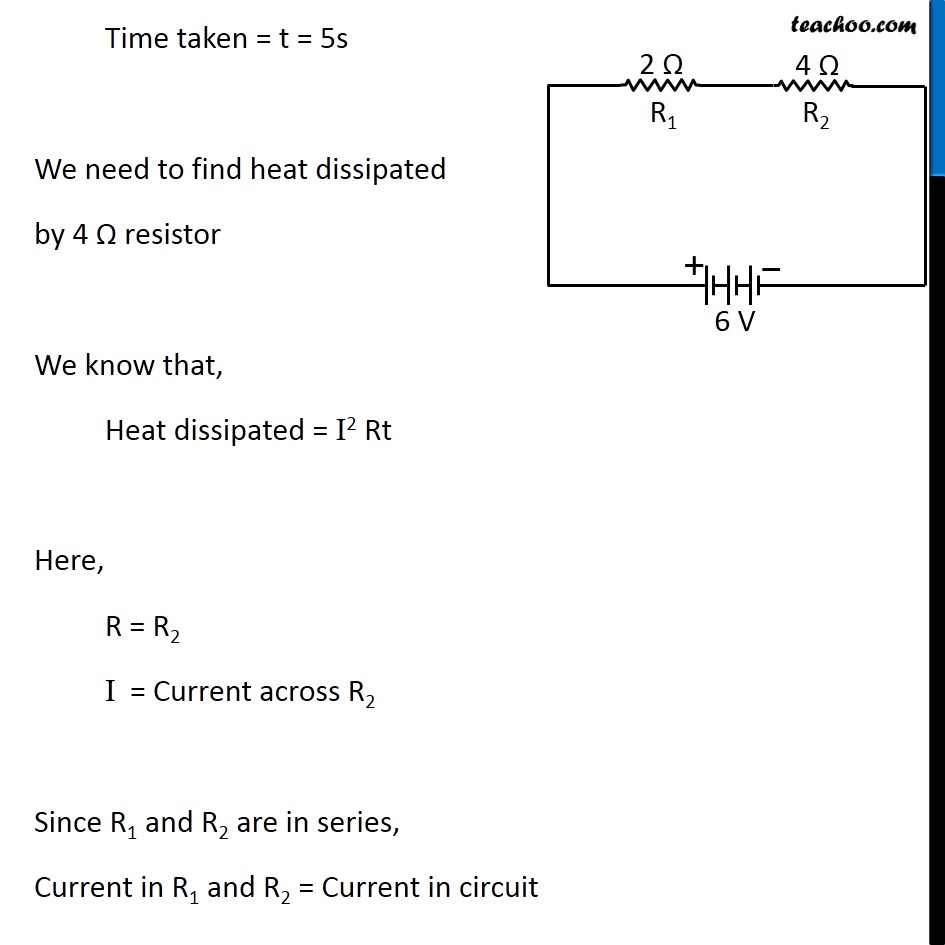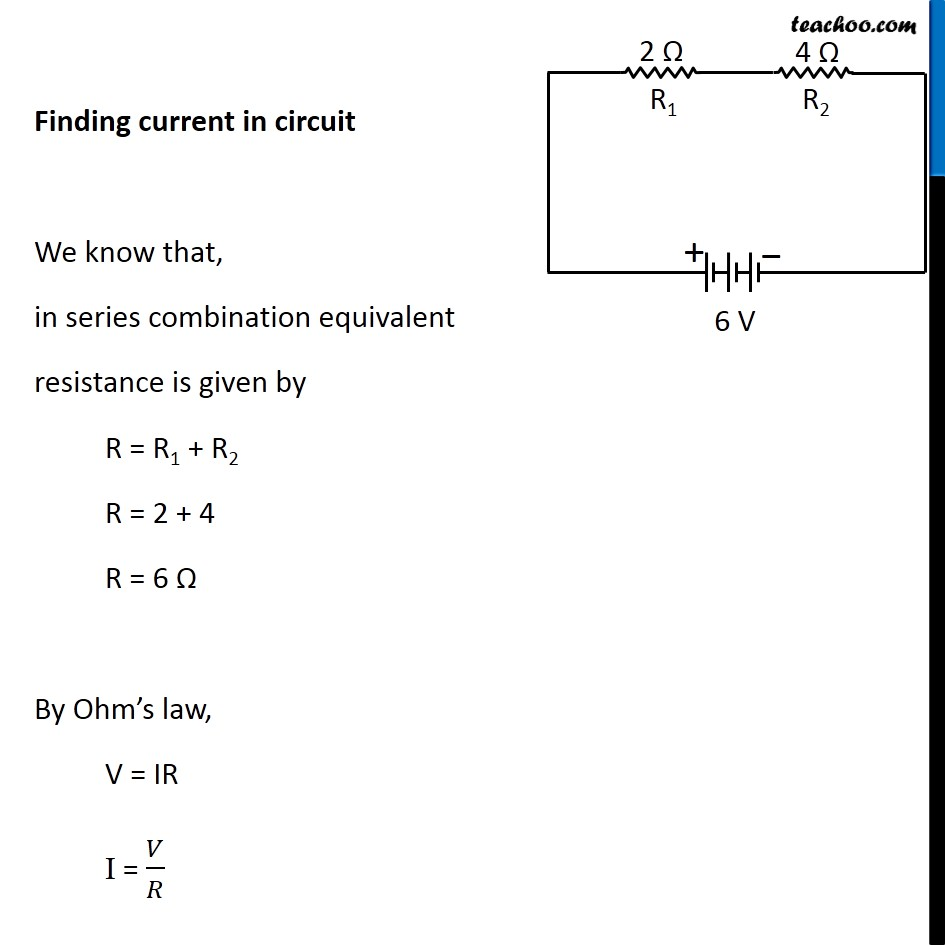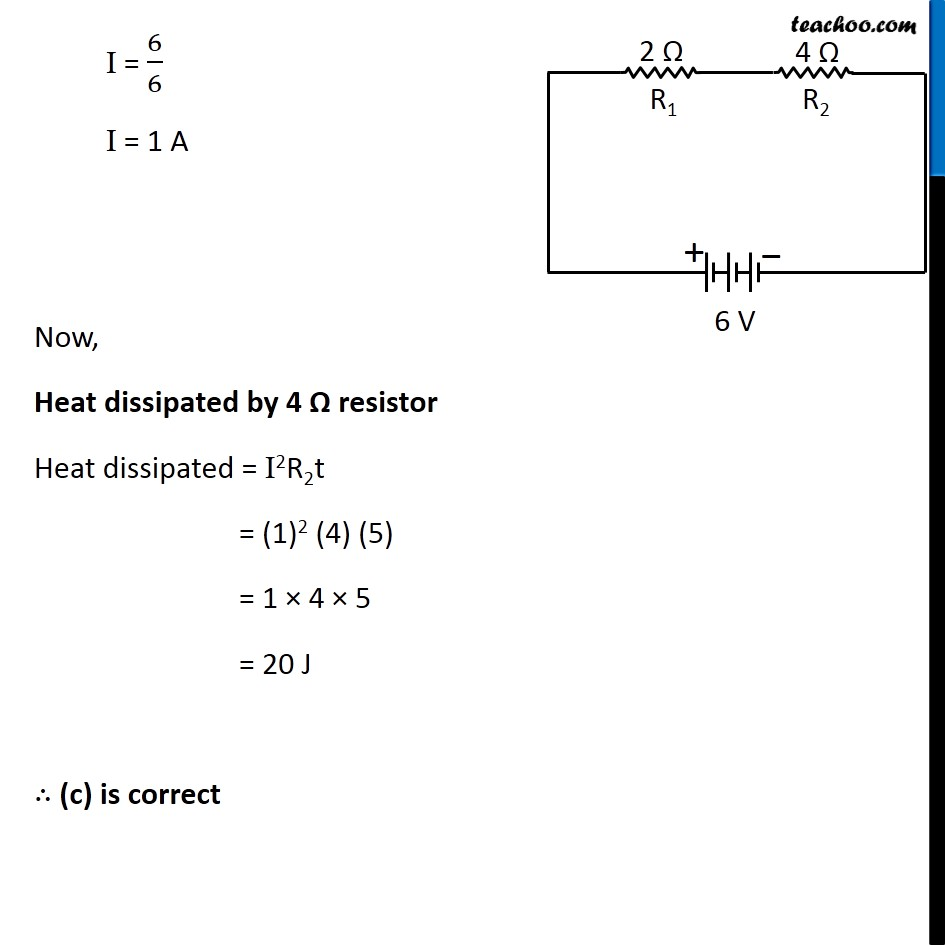## In an electrical circuit two resistors of 2 Ω and 4 Ω respectively are connected in parallel to a 6 V battery. The heat dissipated by the 4 Ω resistor in 5 s will be

(a) 45 J

(b) 20 J

(c) 60 J

(d) 35 J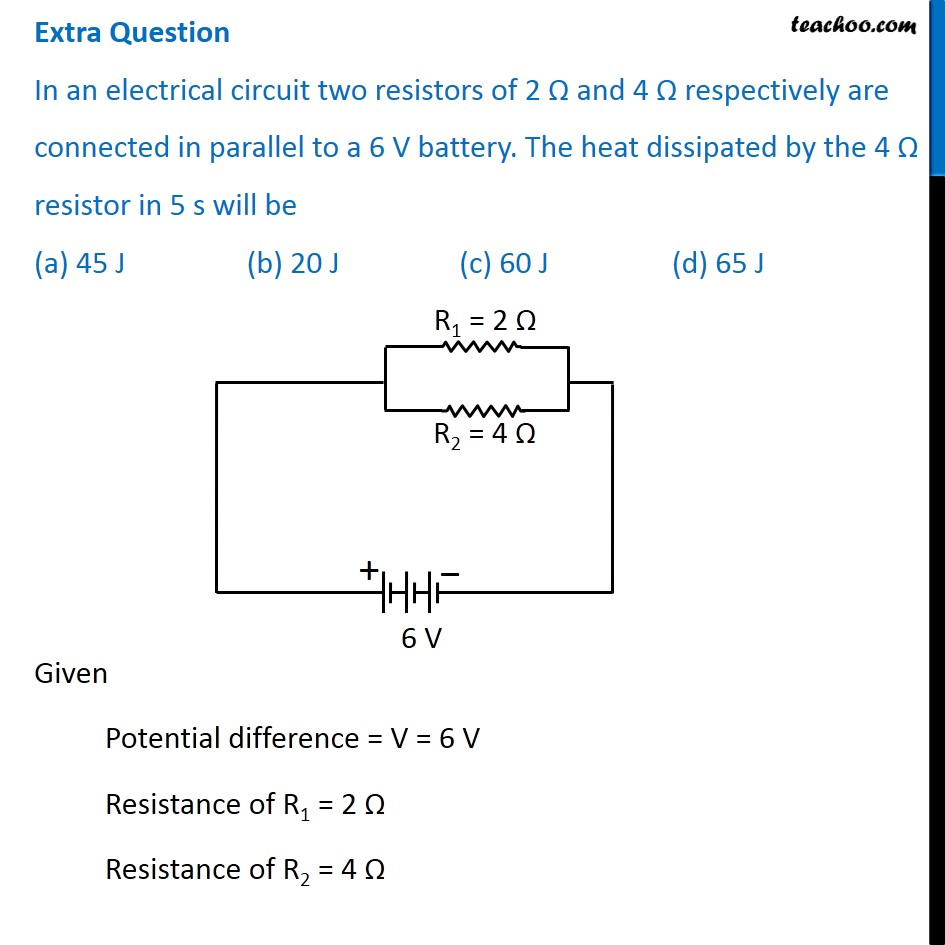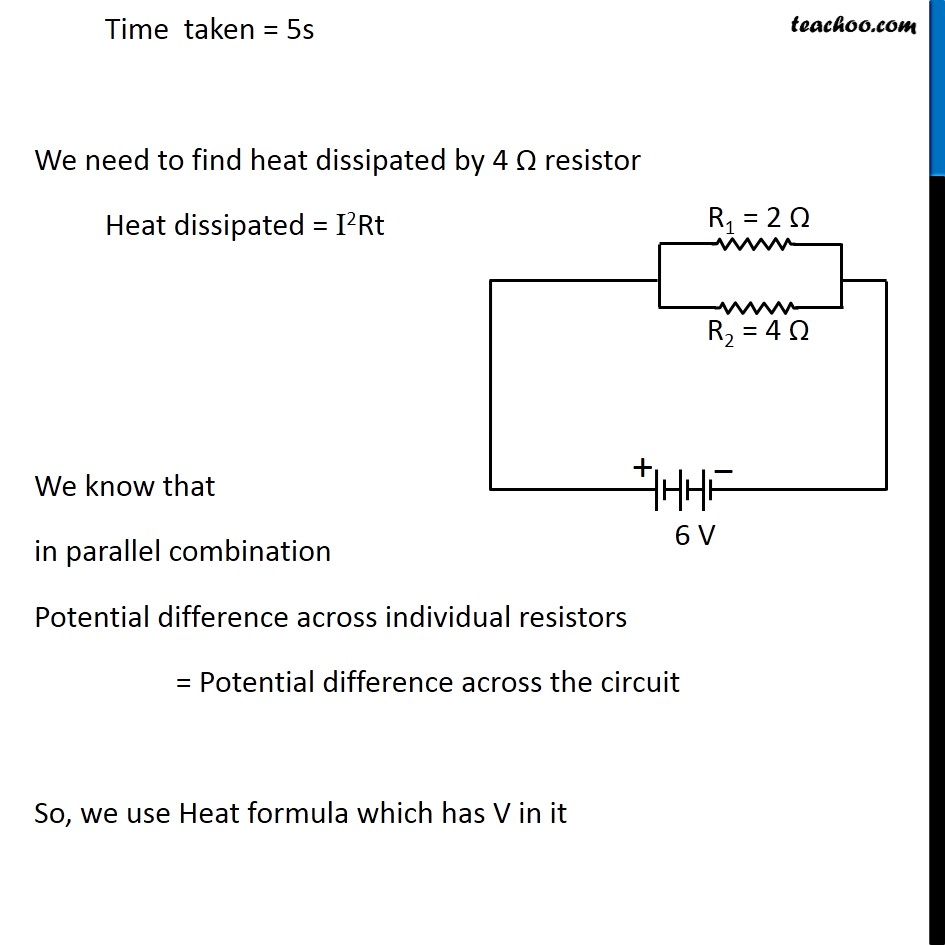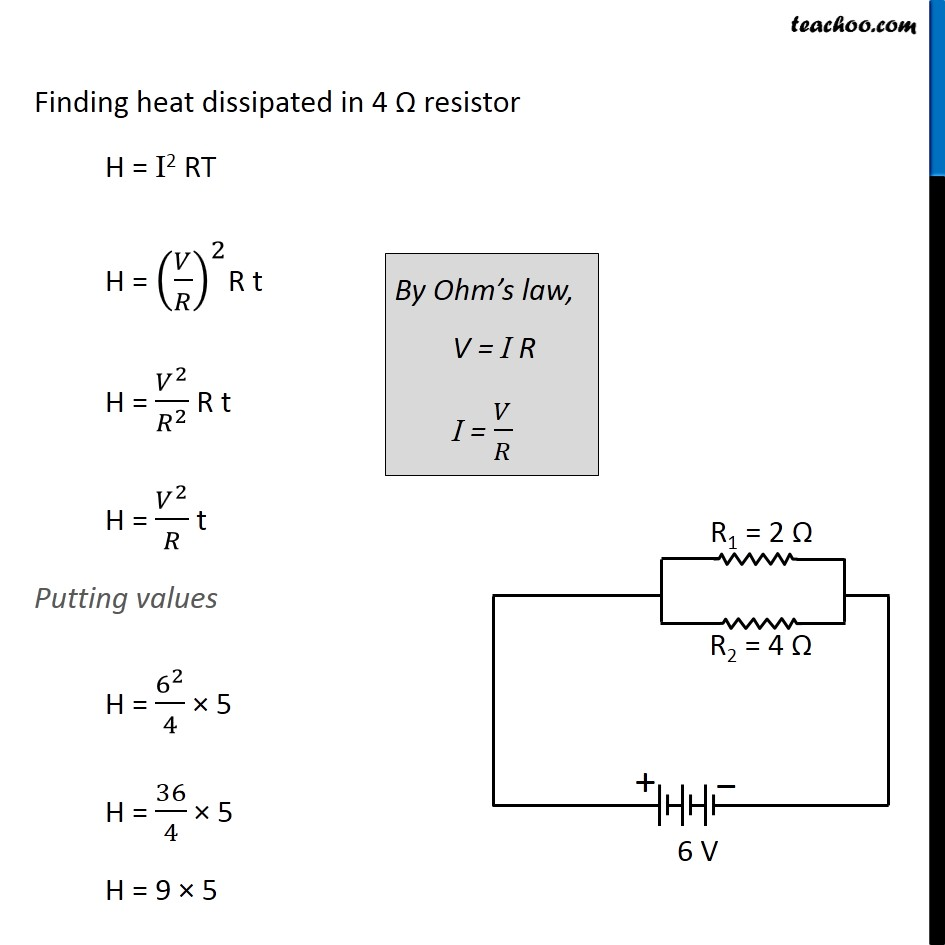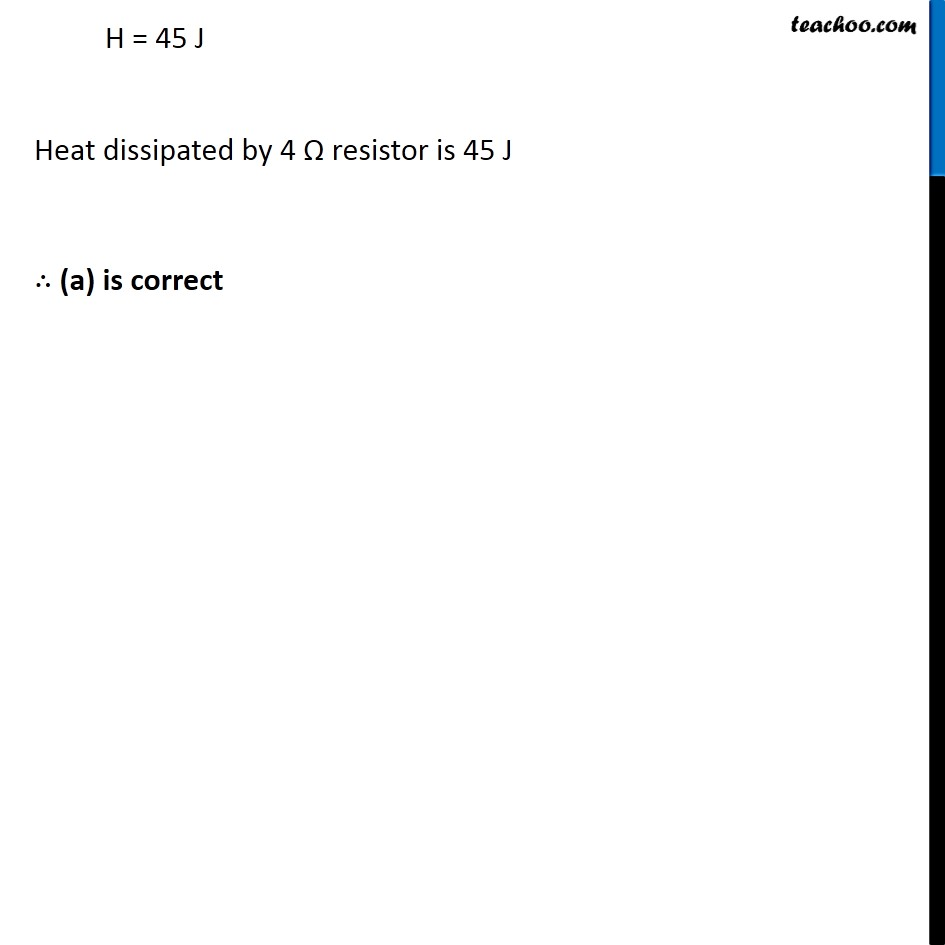## A cell, a resistor, a key and ammeter are arranged as shown in the circuit diagrams below. The current recorded in the ammeter will be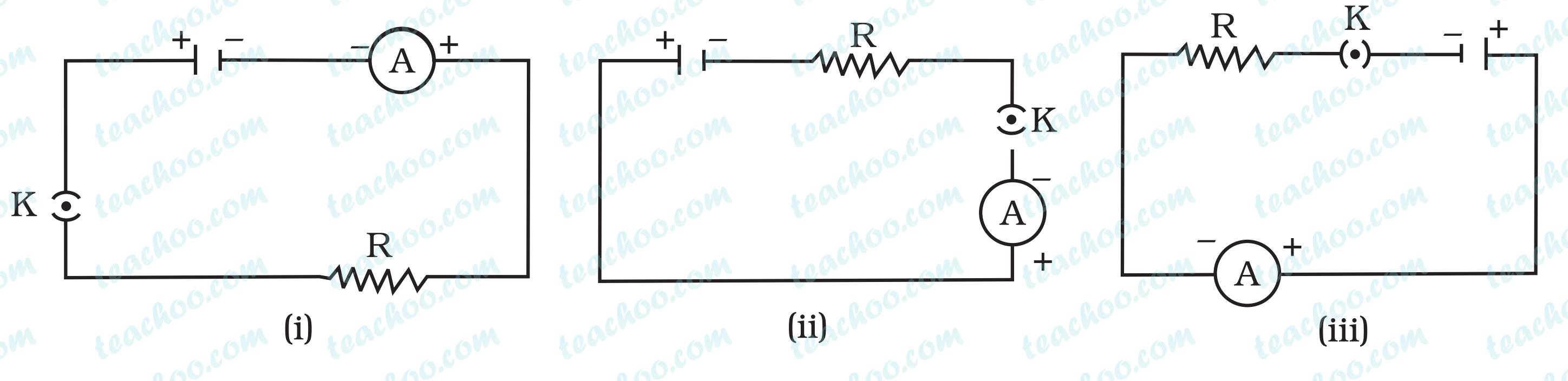(a) maximum in (i)

(b) maximum in (ii)

(c) maximum in (iii)

(d) the same in all the cases

(d) the same in all the cases

Explanation - The current flowing in a circuit does not depend upon the arrangement of the different components, unless they are connected in a different way (Series is changed into parallel, or vice versa.

## A child has drawn the electric circuit to study Ohm’s law as shown in Figure 12.6. His teacher told that the circuit diagram needs correction. Study the circuit diagram and redraw it after making all corrections.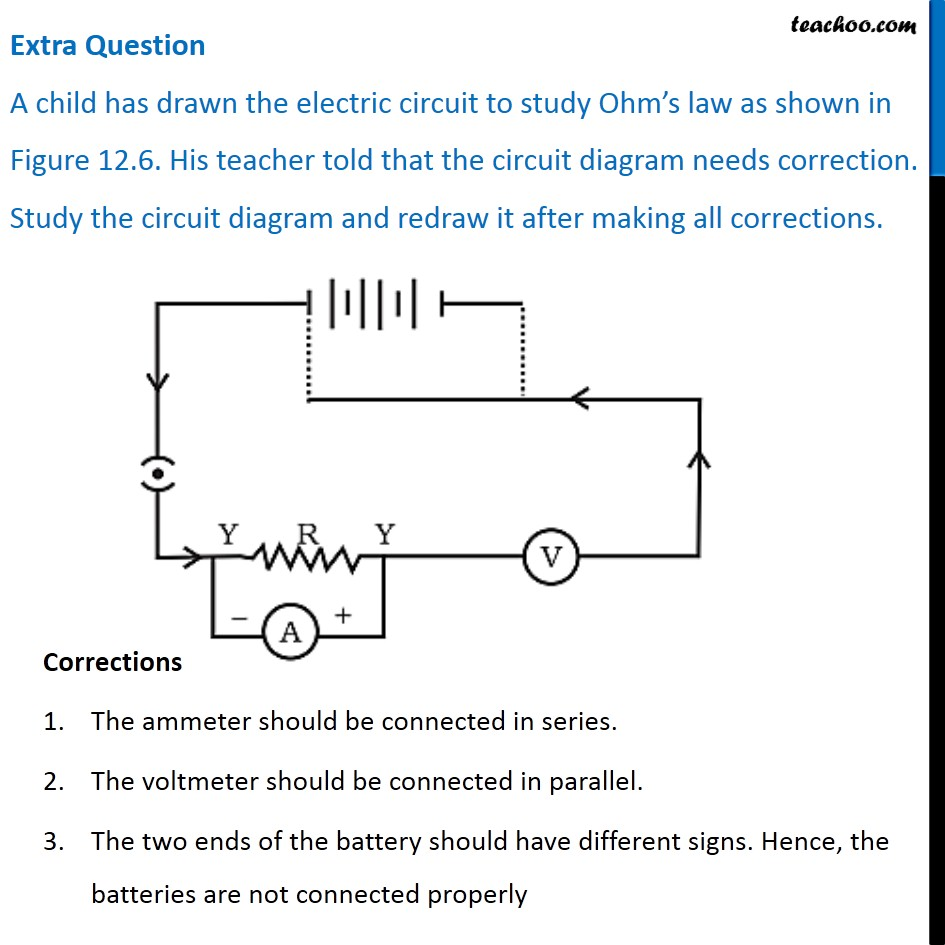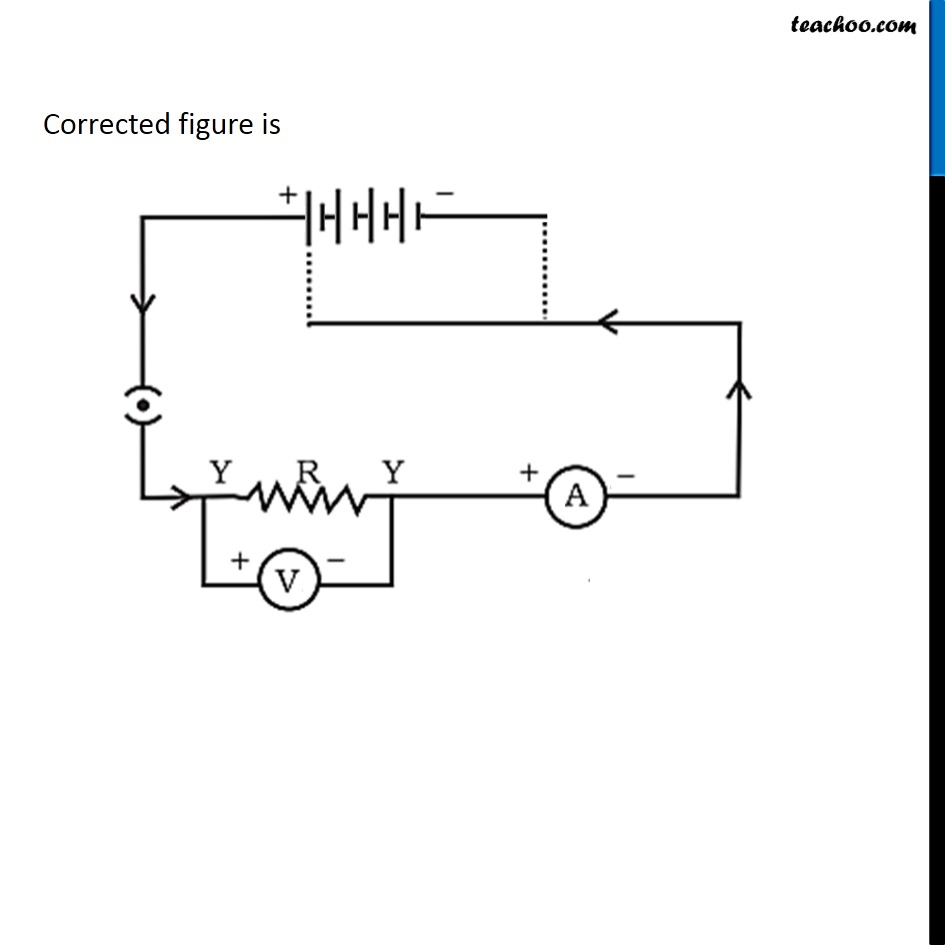## Draw a circuit diagram of an electric circuit containing a cell, a key, an ammeter, a resistor of 2 Ω in series with a combination of two resistors (4 Ω each) in parallel and a voltmeter across the parallel combination. Will the potential difference across the 2 Ω resistor be the same as that across the parallel combination of 4Ω resistors? Give reason.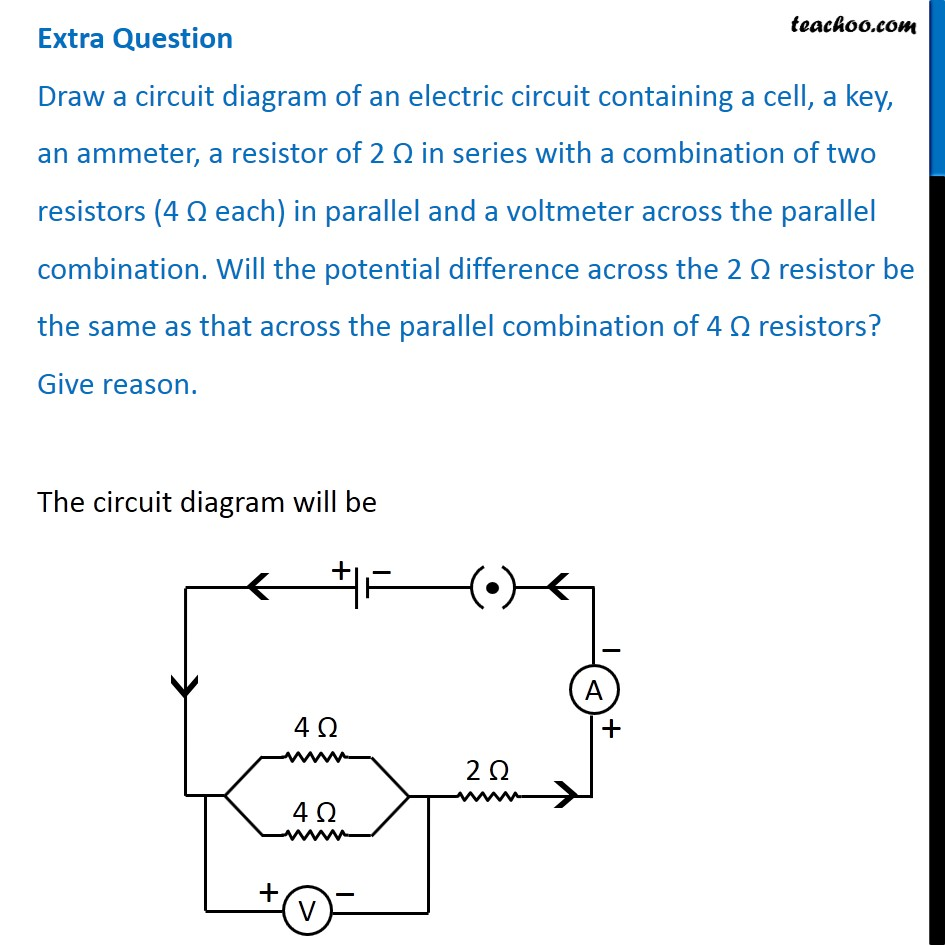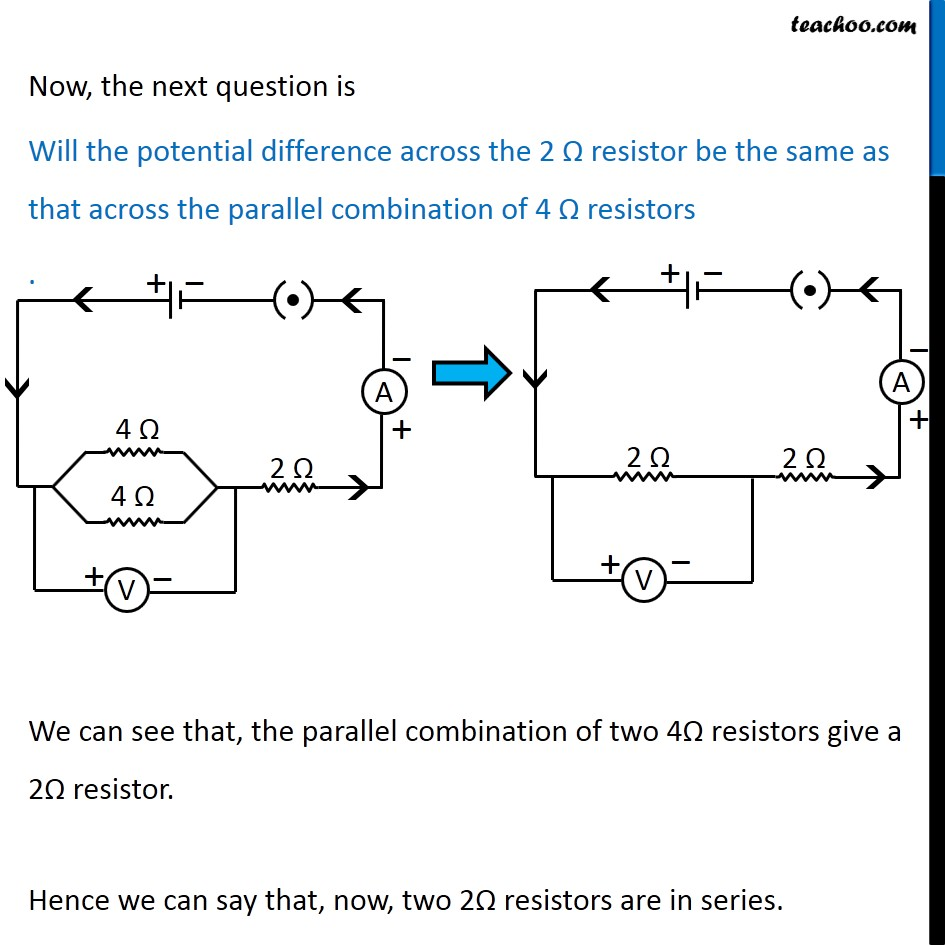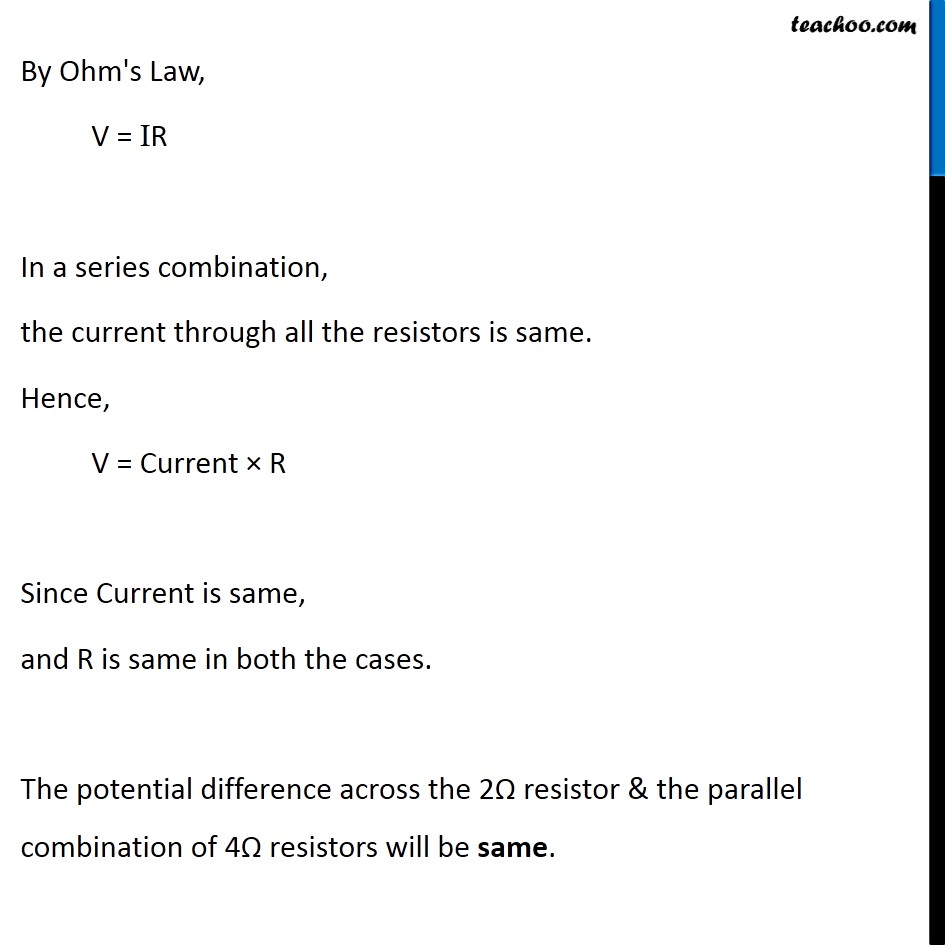## Find out the following in the electric circuit given in Figure 12.9

(a) Effective resistance of two 8 W resistors in the combination

(b) Current flowing through 4 W resistor

(c) Potential difference across 4 W resistance

(d) Power dissipated in 4 W resistor

(e) Difference in ammeter readings, if any.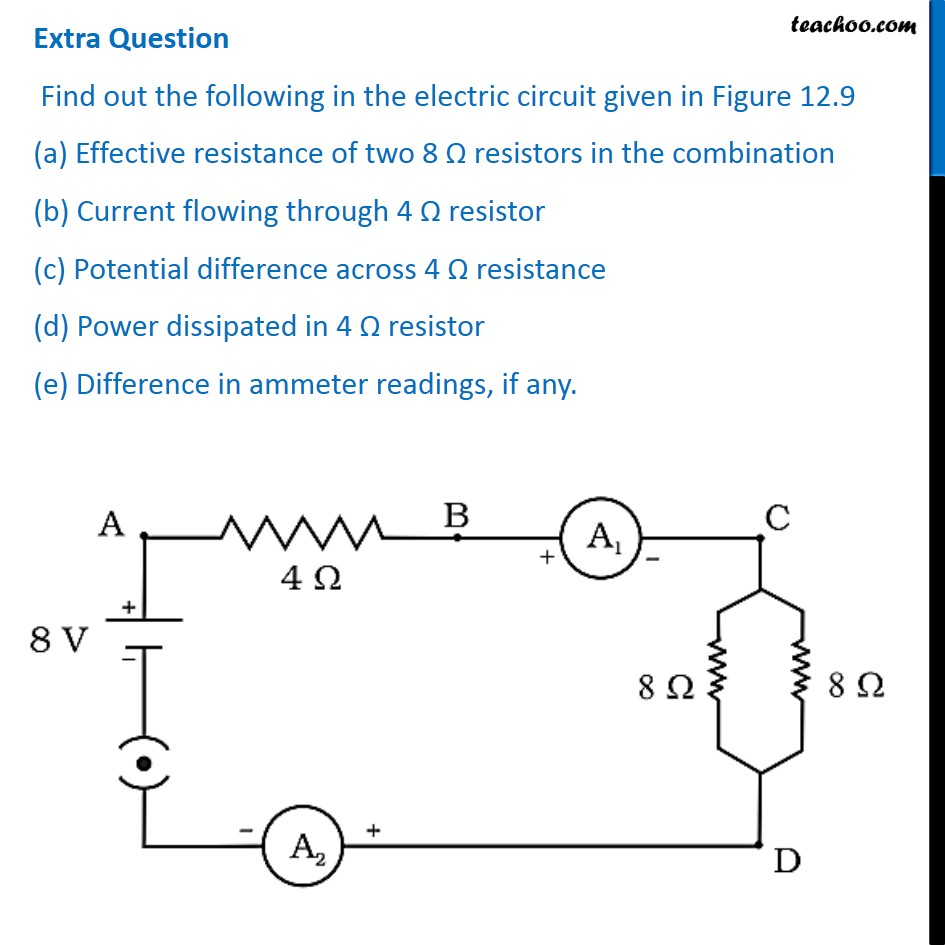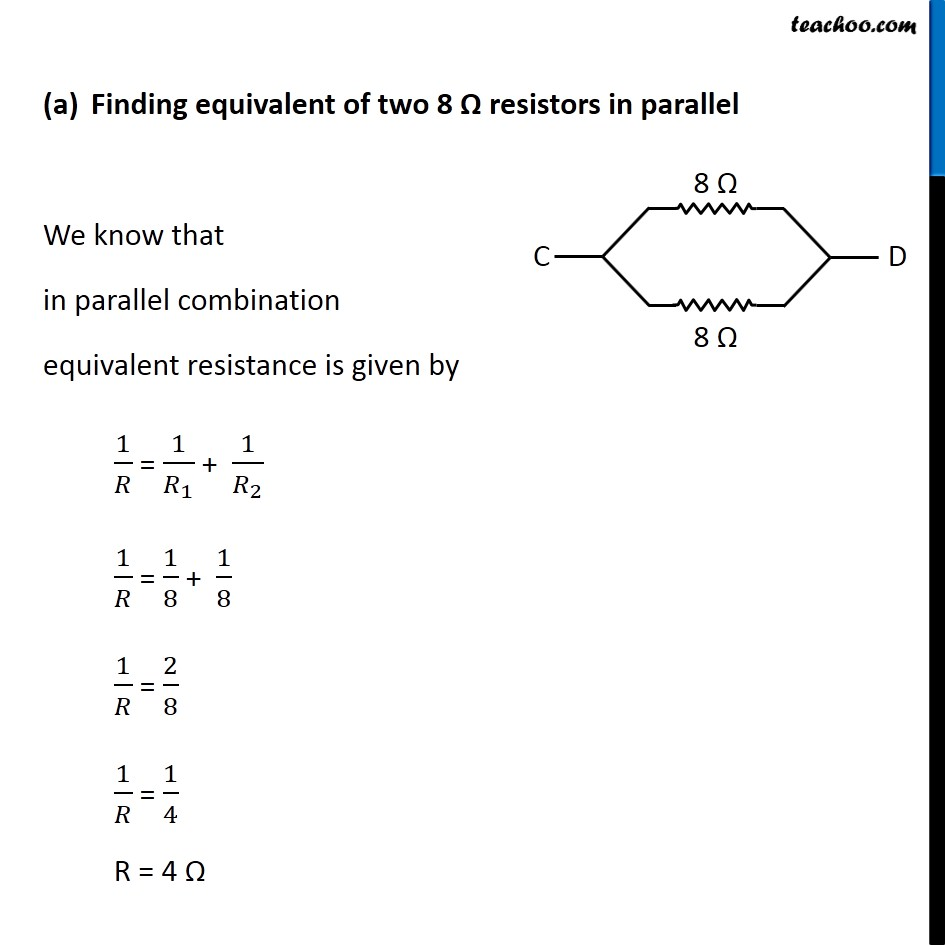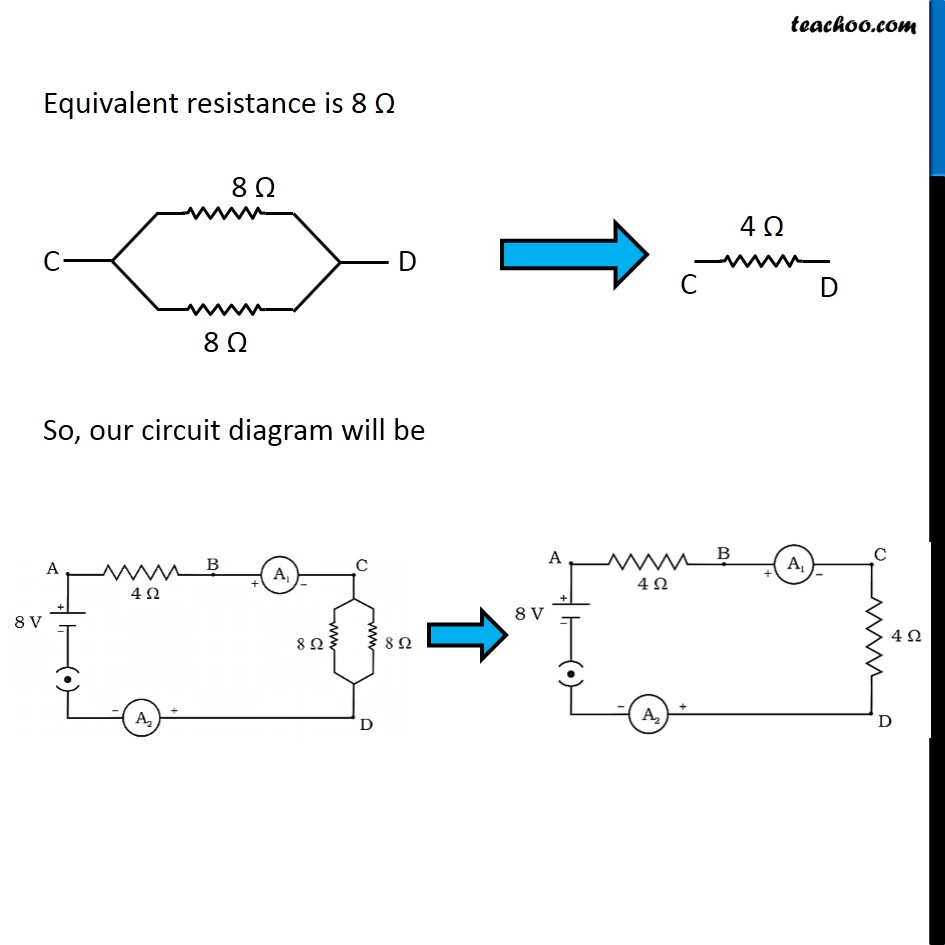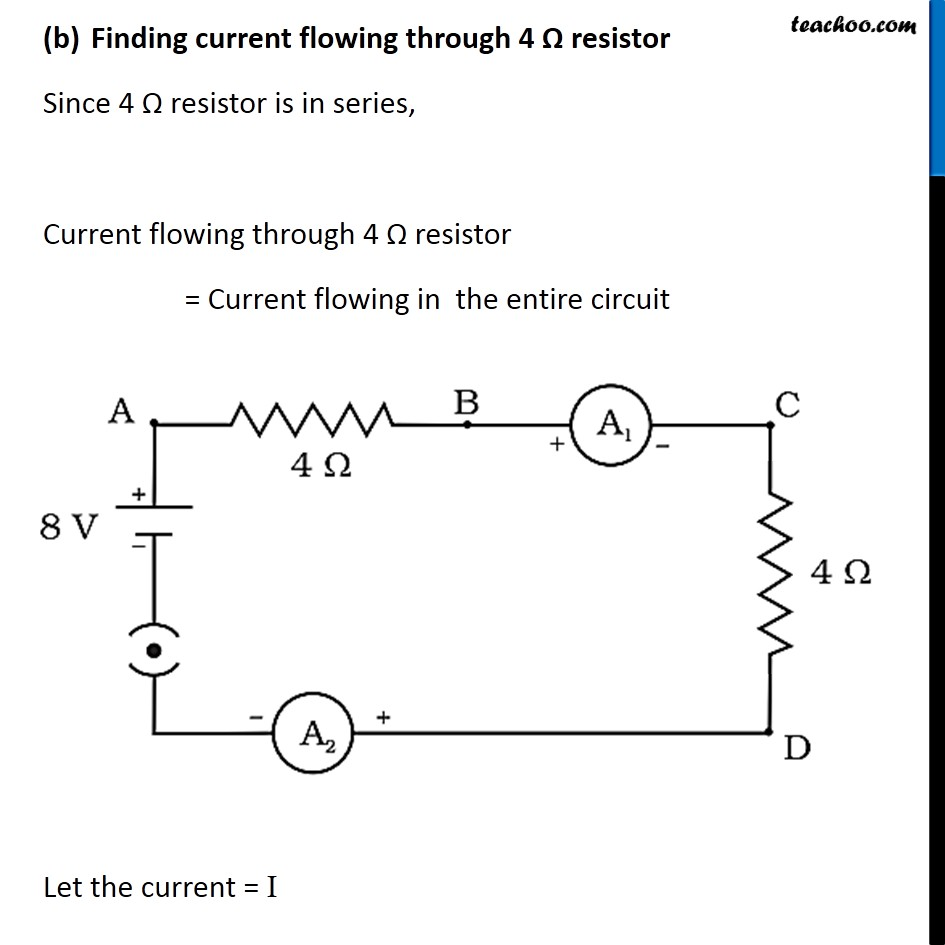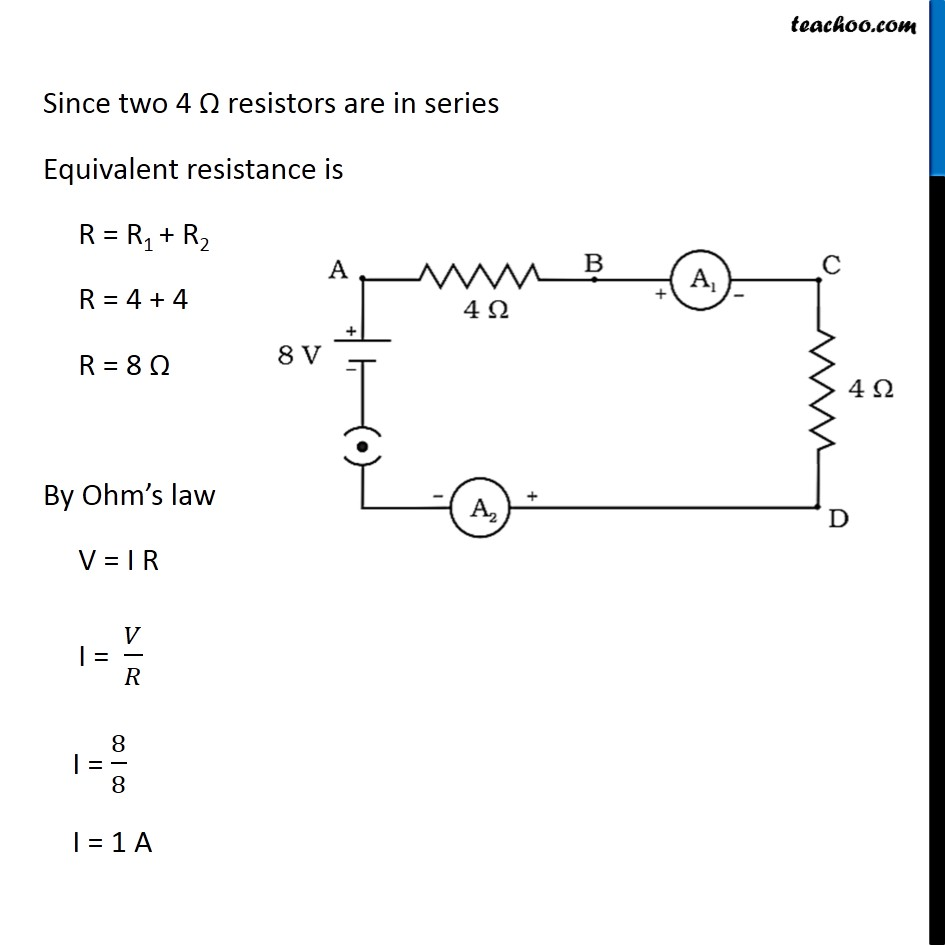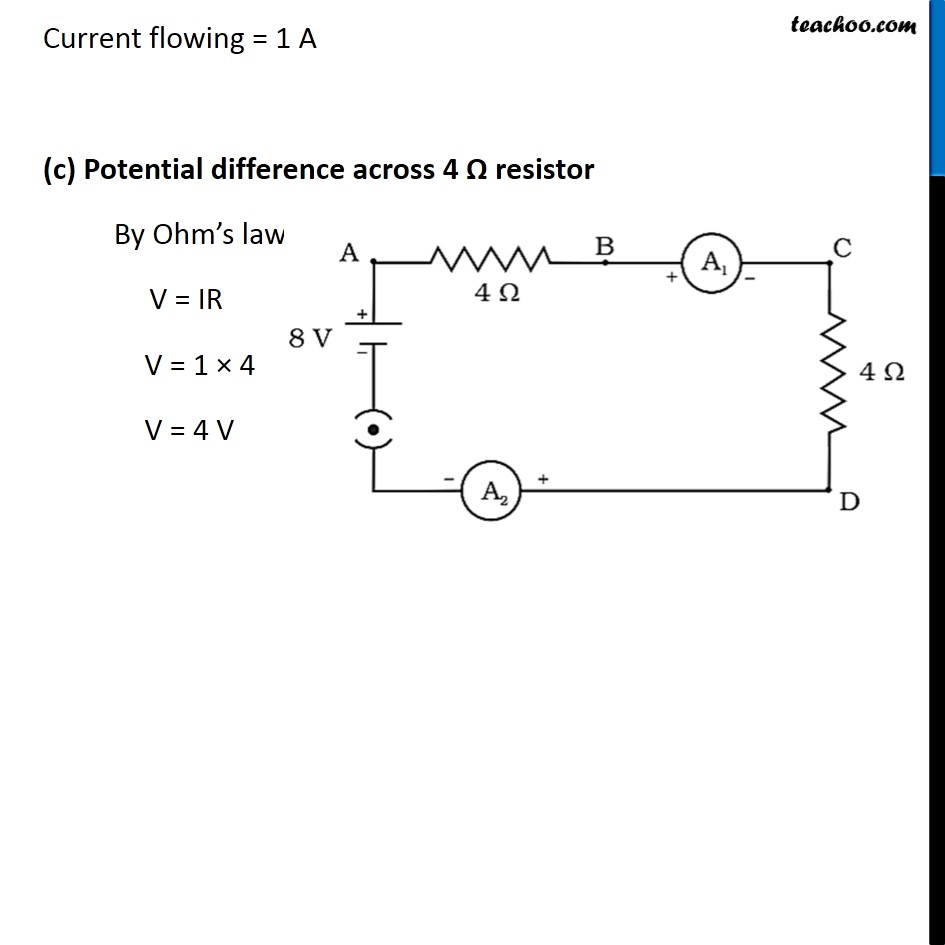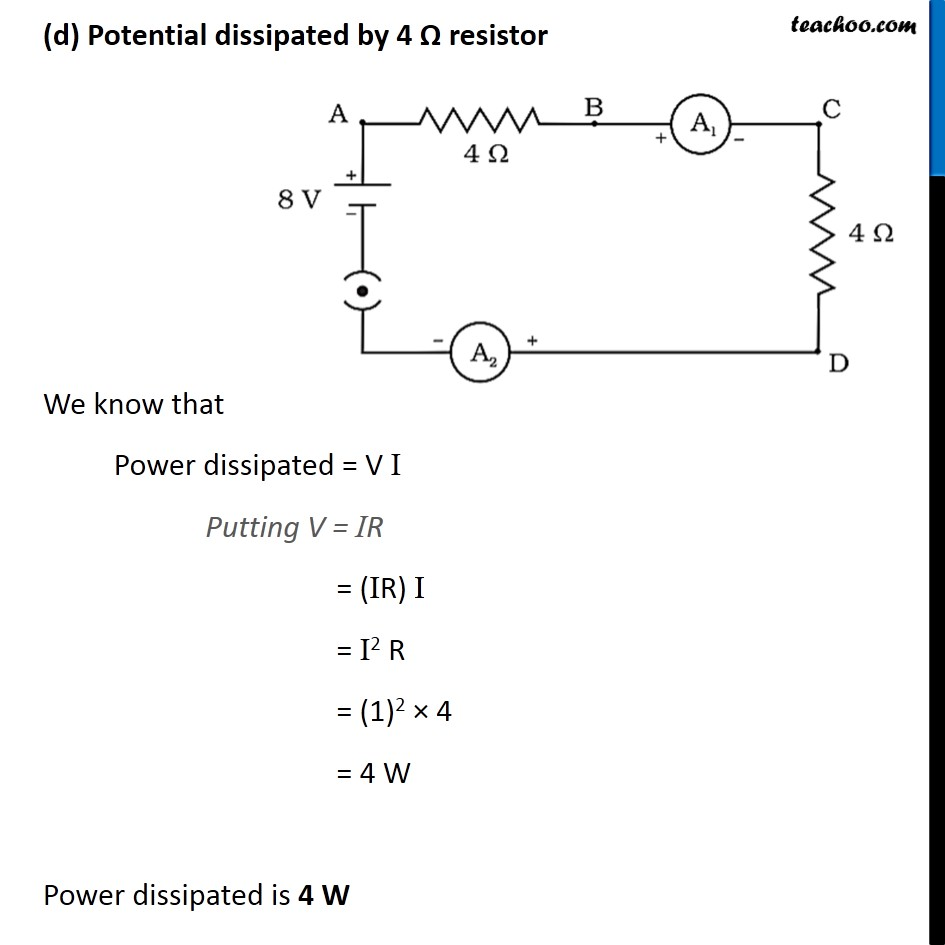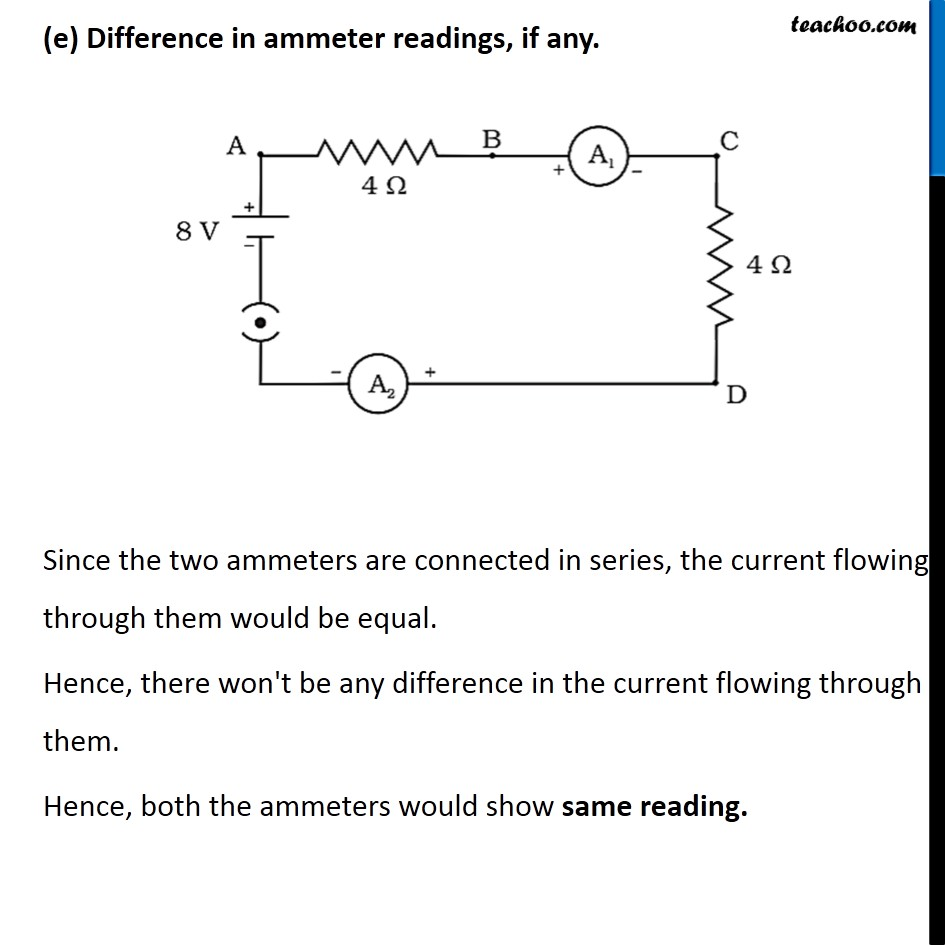## The figure below shows three cylindrical copper conductors along with their face areas and lengths. Compare the resistance and the resistivity of the three conductors. Justify your answer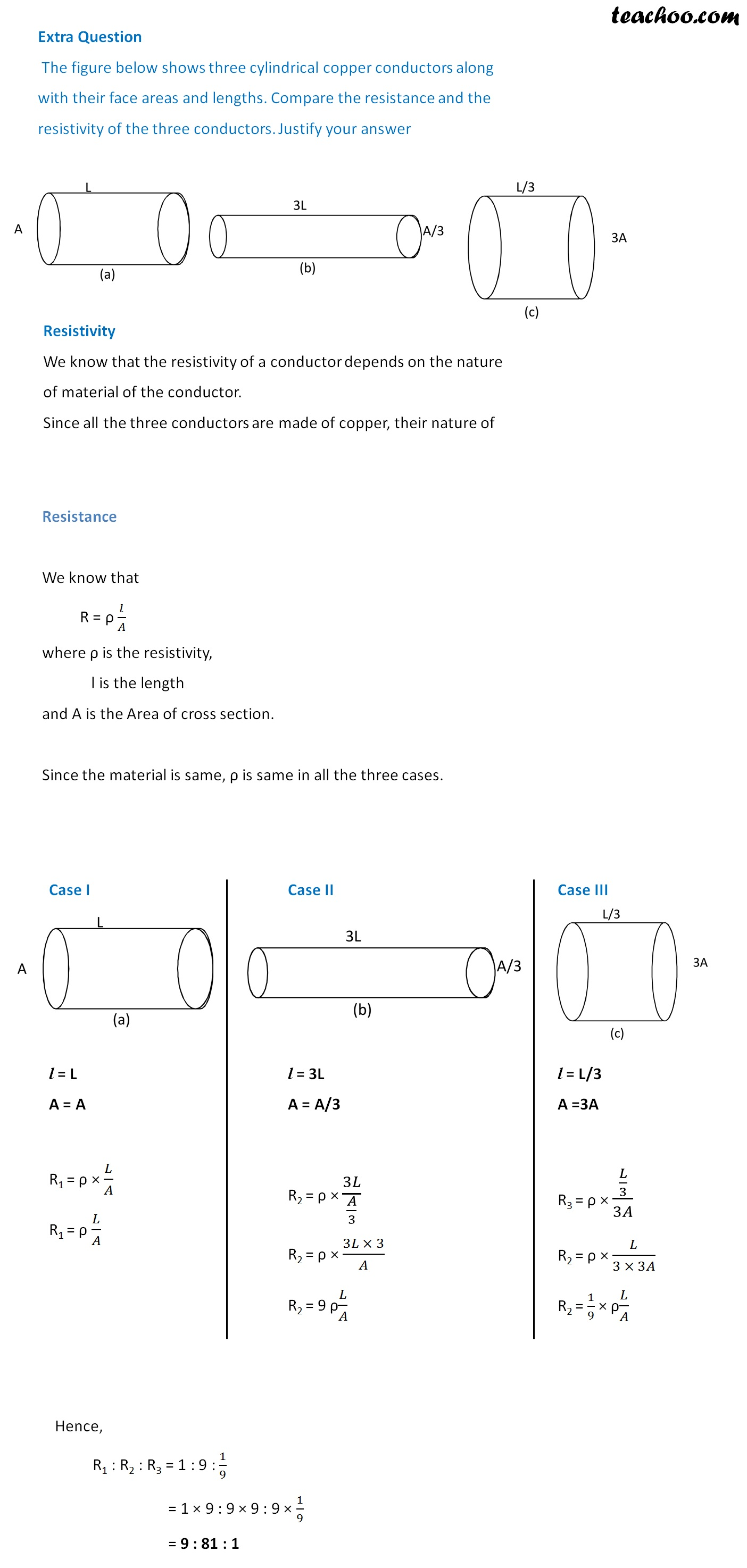## The current flowing through a resistor connected in an electrical circuit and the potential difference developed across its ends are shown in the given ammeter and voltmeter. Find the least count of the voltmeter and ammeter .What is the voltage and the current across the given resistor?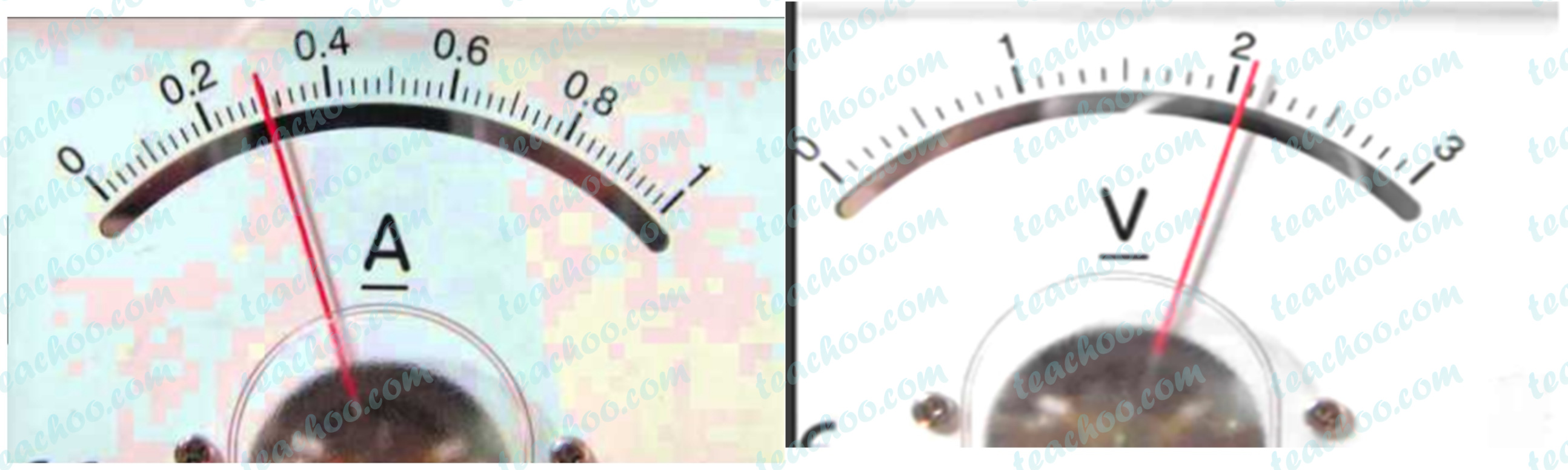### Finding least count

The smallest value that can be measured by a measuring instrument is called its least count.

Numerically,

Least count = Value measure in N divisions/ N

 Ammeter Voltmeter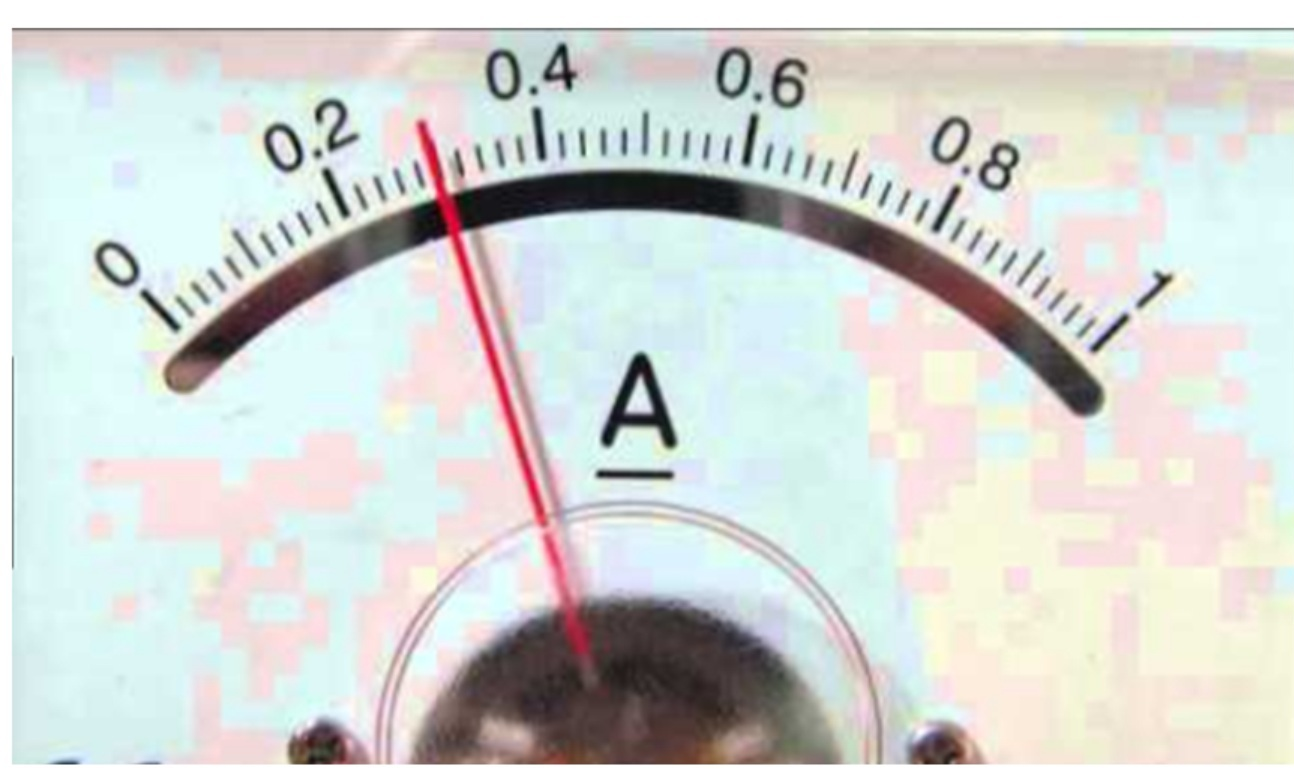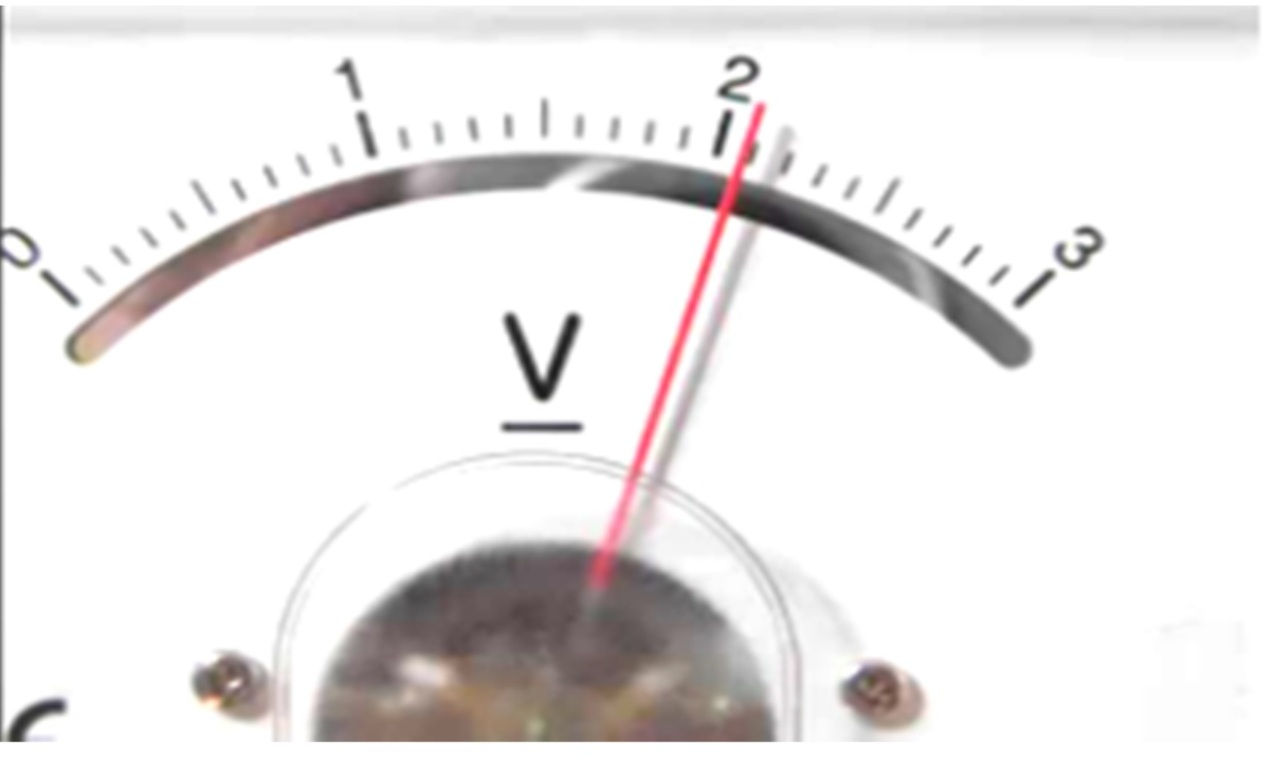We can see that, 10 divisions measure 0.2A current   Therefore, Least count = 0.2/10                  = 0.02 A Least count of ammeter is 0.02 A We can see that, 10 divisions measure 1 V potential difference   Therefore, Least count = 1/10                   = 0.1 V Least count of voltmeter is 0.1 V

### Finding Current through the circuit

The current through the circuit is given by,

Current = Number of division the ammeter needle is pointing x least count

In the given figure, The ammeter needle is pointing on the 15th divison

Hence,

Current = Number of division the ammeter needle is pointing x least count

= 15 × 0.02

= 15 × 2 / 100

= 30 / 100

= 3/10

= 0.3 A

The ammeter reading is 0.3 A.

### Finding potential difference in the circuit

The potential difference in the circuit is given by,

Potential difference = Number of division the voltmeter needle is pointing x least count

In the given figure, The voltmeter needle is pointing on the 21st divison

Hence,

Potential difference = Number of division the ammeter needle is pointing x least count

= 21 × 0.1

= 21 × 1/10

= 21/10

= 2.1 V

## In a given ammeter, a student sees that needle indicates 17 divisions in ammeter while performing an experiment to verify Ohm’s law. If ammeter has 10 divisions between 0 and 0.5A, then what is the value corresponding to 17 divisions?

The current through the circuit is given by,

Current = Number of division the ammeter needle is pointing × least count

The smallest value that can be measured by a measuring instrument is called its least count.

Numerically,

Least count = Value measure in N divisions/ N

According to the question,

There are 10 divisions between 0 and 0.5A

Hence, 10 divisions measure 0.5A current

Therefore,

Least count = Value measure in N divisions/ N

Least count = 0.5/10

= 5/ 10 × 10

= 5/ 100

= 0.05 A

Least count of ammeter is 0.05 A

The current through the circuit is given by,

Current = Number of division the ammeter needle is pointing x least count

According to the question, The ammeter needle is pointing on the 17th divison

Hence,

Current = Number of division the ammeter needle is pointing × least count

= 17 × 0.05

= 17 × 5 / 100

= 85 / 100

= 0.85 A

The ammeter reading is 0.85 A.

Get live Maths 1-on-1 Classs - Class 6 to 12

### Transcript

Extra Question A current of 1 A is drawn by a filament of an electric bulb. Number of electrons passing through a cross section of the filament in 16 seconds would be roughly (a) 1020 (b) 1016 (c) 1018 (d) 1023 Current drawn = I = 1 A Time taken = t = 16 s We need to find number of electrons passing First, we need to find the change flown Let the change flown = Q We know that I = 𝑄/𝑡 Q = I t Q = 1 × 16 Q = 16 C We know that Charge on 1 electron = 1.6 × 10−19 C Therefore, 1.6 × 10−19 C = 1 electrons 1 C = 1/(1.6 × 〖10〗^(−19) ) electrons 1 C = 〖10〗^19/1.6 electrons 1 C = 〖10〗^19/16 × 10 electrons 1 C = (〖10〗^20 )/16 electrons Therefore, Number of electrons is 1 C charge = 〖10〗^20/16 electrons Number of electrons is 16 C charge = 16 × 〖10〗^20/16 electrons = 1020 electrons ∴ (a) is correct Extra Question What is the maximum resistance which can be made using five resistors each of 1/5 Ω? (a) 1/5 Ω (b) 10 Ω (c) 5 Ω (d) 1 Ω Resistance of each resistors = 1/5 Ω Number of resistors = 5 We know that, equivalent resistance is maximum when resistors are in series combination We know that, equivalent resistance is given by R = R1 + R2 + R3 + R4 + R5 R = 1/5 + 1/5 + 1/5 + 1/5 + 1/5 R = 5/5 R = 1 Ω Maximum resistance is 1 Ω ∴ (d) is correct Extra Question What is the minimum resistance which can be made using five resistors each of 1/5 Ω? (a) 1/5 Ω (b) 1/25 Ω (c) 1/10 Ω (d) 25 Ω Resistance of each resistors = 1/5 Ω Number of resistors = 5 We know that, equivalent resistance is minimum when resistors are in parallel combination We know that, equivalent resistance is given by R = R1 + R2 + R3 + R4 + R5 1/𝑅 = 1/(1/5) + 1/(1/5) + 1/(1/5) + 1/(1/5) + 1/(1/5) 1/𝑅 = 5/1 + 5/1 + 5/1 + 5/1 + 5/1 1/𝑅 = 25 R = 1/25 Ω Minimum resistance is 𝟏/𝟐𝟓 Ω ∴ (b) is correct Extra Question A cylindrical conductor of length l and uniform area of cross section A has resistance R. Another conductor of length 2l and resistance R of the same material has area of cross section (a) A/2 (b) 3A/2 (c) 2 A (d) 3 A Given Length of the conductor = l Area of cross-section = A Resistance of the conductor = R Let the resistivity of the conductor = 𝜌 We know that, R = 𝜌 𝑙/𝐴 ∴ A = 𝝆𝒍/𝑹 New Conductor Given, Length of other conductor = l2 = 2l Resistance of the conductor = R Since the material is same, Resistivity of the conductor = 𝜌 Now, R = 𝜌 𝑙_2/𝐴_2 R = 𝜌 × 2𝑙/𝐴_2 A2 = (𝜌 (2𝑙))/𝑅 A2 = 2((𝜌 𝑙)/𝑅) A2 = 2 A ∴ (c) is correct Extra Question In an electrical circuit two resistors of 2 Ω and 4 Ω respectively are connected in series to a 6 V battery. The heat dissipated by the 4 Ω resistor in 5 s will be (a) 5 J (b) 10 J (c) 20 J (d) 30 J Given Potential difference = V = 6 V Resistance of R1 = 2 Ω Resistance of R2 = 4 Ω Time taken = t = 5s We need to find heat dissipated by 4 Ω resistor We know that, Heat dissipated = I2 Rt Here, R = R2 I = Current across R2 Since R1 and R2 are in series, Current in R1 and R2 = Current in circuit Finding current in circuit We know that, in series combination equivalent resistance is given by R = R1 + R2 R = 2 + 4 R = 6 Ω By Ohm’s law, V = IR I = 𝑉/𝑅 I = 6/6 I = 1 A Now, Heat dissipated by 4 Ω resistor Heat dissipated = I2R2t = (1)2 (4) (5) = 1 × 4 × 5 = 20 J ∴ (c) is correct Extra Question In an electrical circuit two resistors of 2 Ω and 4 Ω respectively are connected in parallel to a 6 V battery. The heat dissipated by the 4 Ω resistor in 5 s will be (a) 45 J (b) 20 J (c) 60 J (d) 65 J Given Potential difference = V = 6 V Resistance of R1 = 2 Ω Resistance of R2 = 4 Ω Time taken = 5s We need to find heat dissipated by 4 Ω resistor Heat dissipated = I2Rt We know that in parallel combination Potential difference across individual resistors = Potential difference across the circuit So, we use Heat formula which has V in it Finding heat dissipated in 4 Ω resistor H = I2 RT H = (𝑉/𝑅)^2R t H = 𝑉^2/𝑅^2 R t H = 𝑉^2/𝑅 t Putting values H = 6^2/4 × 5 H = 36/4 × 5 H = 9 × 5 By Ohm’s law, V = I R I = 𝑉/𝑅 H = 45 J Heat dissipated by 4 Ω resistor is 45 J ∴ (a) is correct Extra Question A child has drawn the electric circuit to study Ohm’s law as shown in Figure 12.6. His teacher told that the circuit diagram needs correction. Study the circuit diagram and redraw it after making all corrections. Corrections The ammeter should be connected in series. The voltmeter should be connected in parallel. The two ends of the battery should have different signs. Hence, the batteries are not connected properly Corrected figure is Extra Question Draw a circuit diagram of an electric circuit containing a cell, a key, an ammeter, a resistor of 2 Ω in series with a combination of two resistors (4 Ω each) in parallel and a voltmeter across the parallel combination. Will the potential difference across the 2 Ω resistor be the same as that across the parallel combination of 4 Ω resistors? Give reason. The circuit diagram will be Now, the next question is Will the potential difference across the 2 Ω resistor be the same as that across the parallel combination of 4 Ω resistors . We can see that, the parallel combination of two 4Ω resistors give a 2Ω resistor. Hence we can say that, now, two 2Ω resistors are in series. By Ohm's Law, V = IR In a series combination, the current through all the resistors is same. Hence, V = Current × R Since Current is same, and R is same in both the cases. The potential difference across the 2Ω resistor & the parallel combination of 4Ω resistors will be same. Extra Question Find out the following in the electric circuit given in Figure 12.9 (a) Effective resistance of two 8 Ω resistors in the combination (b) Current flowing through 4 Ω resistor (c) Potential difference across 4 Ω resistance (d) Power dissipated in 4 Ω resistor (e) Difference in ammeter readings, if any. Finding equivalent of two 8 Ω resistors in parallel We know that in parallel combination equivalent resistance is given by 1/𝑅 = 1/𝑅_1 + 1/𝑅_2 1/𝑅 = 1/8 + 1/8 1/𝑅 = 2/8 1/𝑅 = 1/4 R = 4 Ω Equivalent resistance is 8 Ω So, our circuit diagram will be Finding current flowing through 4 Ω resistor Since 4 Ω resistor is in series, Current flowing through 4 Ω resistor = Current flowing in the entire circuit Let the current = I Since two 4 Ω resistors are in series Equivalent resistance is R = R1 + R2 R = 4 + 4 R = 8 Ω By Ohm’s law V = I R I = 𝑉/𝑅 I = 8/8 I = 1 A Current flowing = 1 A (c) Potential difference across 4 Ω resistor By Ohm’s law, V = IR V = 1 × 4 V = 4 V (d) Potential dissipated by 4 Ω resistor We know that Power dissipated = V I Putting V = IR = (IR) I = I2 R = (1)2 × 4 = 4 W Power dissipated is 4 W (e) Difference in ammeter readings, if any. Since the two ammeters are connected in series, the current flowing through them would be equal. Hence, there won't be any difference in the current flowing through them. Hence, both the ammeters would show same reading. Extra Question The figure below shows three cylindrical copper conductors along with their face areas and lengths. Compare the resistance and the resistivity of the three conductors. Justify your answer Resistivity We know that the resistivity of a conductor depends on the nature of material of the conductor. Since all the three conductors are made of copper, their nature of material is same. Hence, their resistivity will be same. Resistance We know that R = ρ 𝑙/𝐴 where ρ is the resistivity, l is the length and A is the Area of cross section. Since the material is same, ρ is same in all the three cases. Case I l = L A = A R1 = ρ × 𝐿/𝐴 R1 = ρ 𝐿/𝐴 Case II l = 3L A = A/3 R2 = ρ × 3𝐿/(𝐴/3) R2 = ρ × (3𝐿 × 3)/𝐴 R2 = 9 ρ𝐿/𝐴 Case III I = L/3 l = L/3 A =3A R3 = ρ × (𝐿/3)/3𝐴 R2 = ρ × 𝐿/(3 × 3𝐴) R2 = 1/9 × ρ𝐿/𝐴 Hence, R1 : R2 : R3 = 1 : 9 : 1/9 = 1 × 9 : 9 × 9 : 9 × 1/9 = 9 : 81 : 1